# Selina Solutions Concise Maths Class 10 Chapter 17 Circles

A circle is the locus of a point which moves in a plane in such a way that its distance from a fixed point in the same plane always remains constant. In this chapter, students will learn the basic types and parts of a circle, cyclic properties and some important theorems and results. Students wanting to get a clear picture of concepts and problem solving techniques can access the Selina Solutions for Class 10 Mathematics prepared by expert faculty at BYJU’S. The solutions will also boost confidence among students to take up their Class 10 ICSE. The Selina Solutions for Class 10 Mathematics Chapter 17 Circles PDFs are available exercise-wise in the link below.

## Selina Solutions Concise Maths Class 10 Chapter 17 Circles Download PDF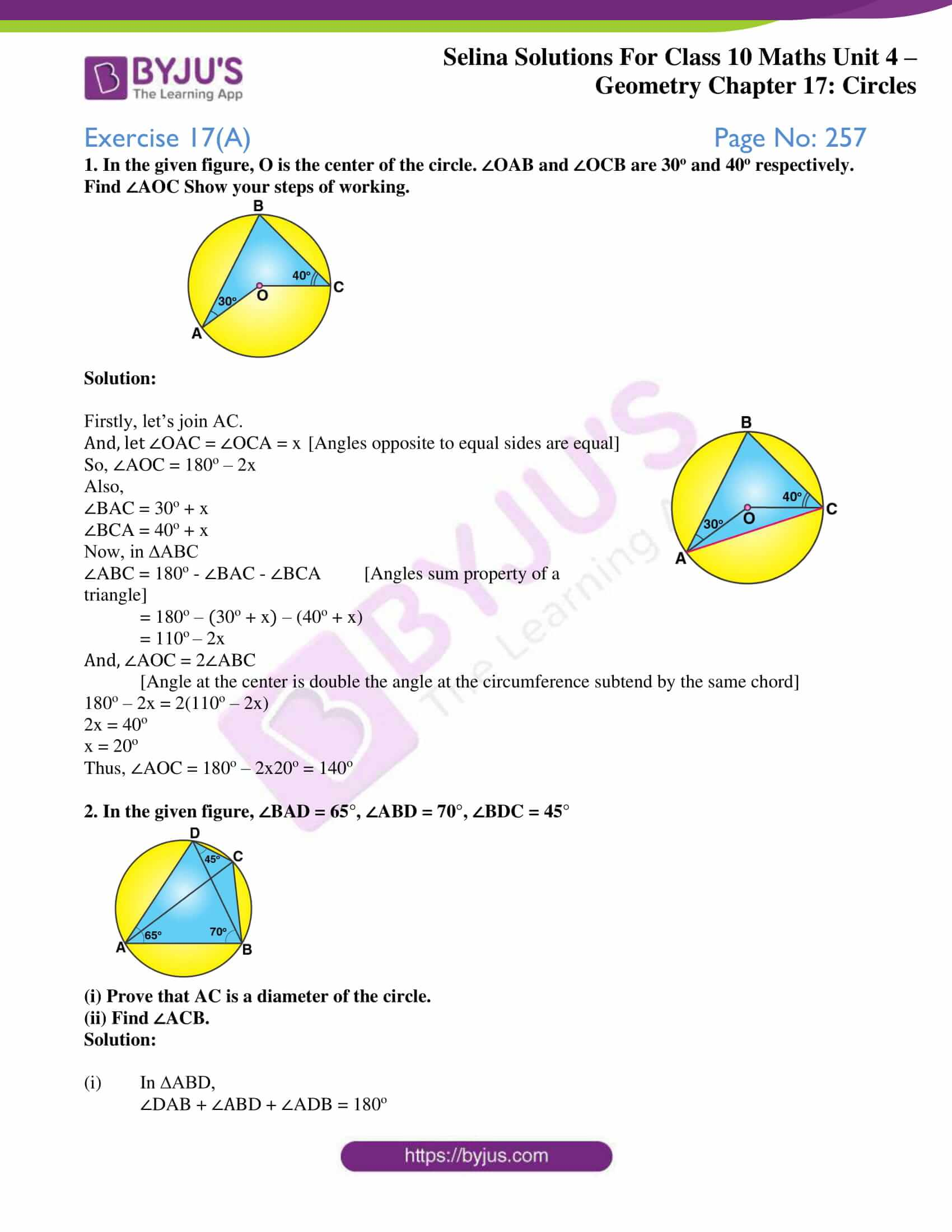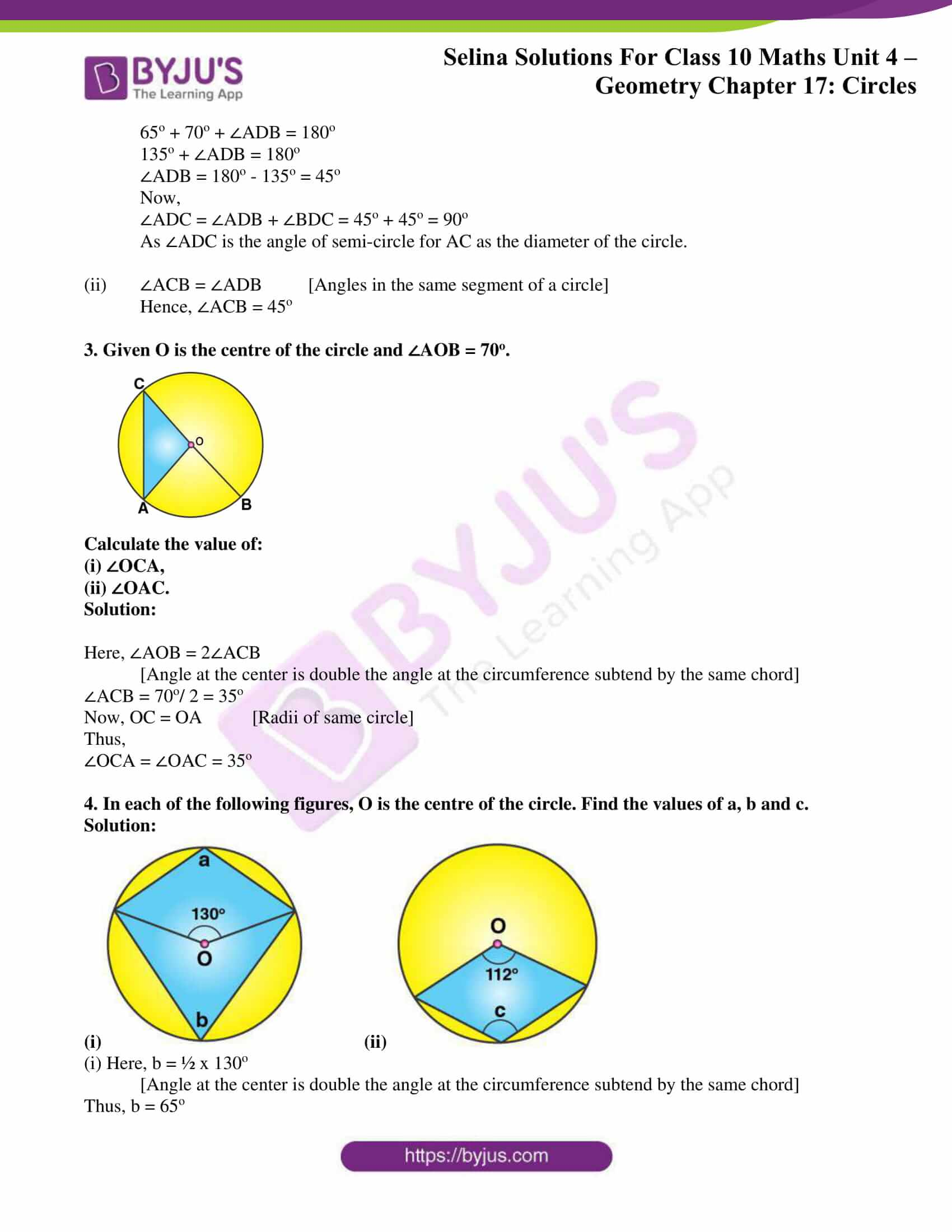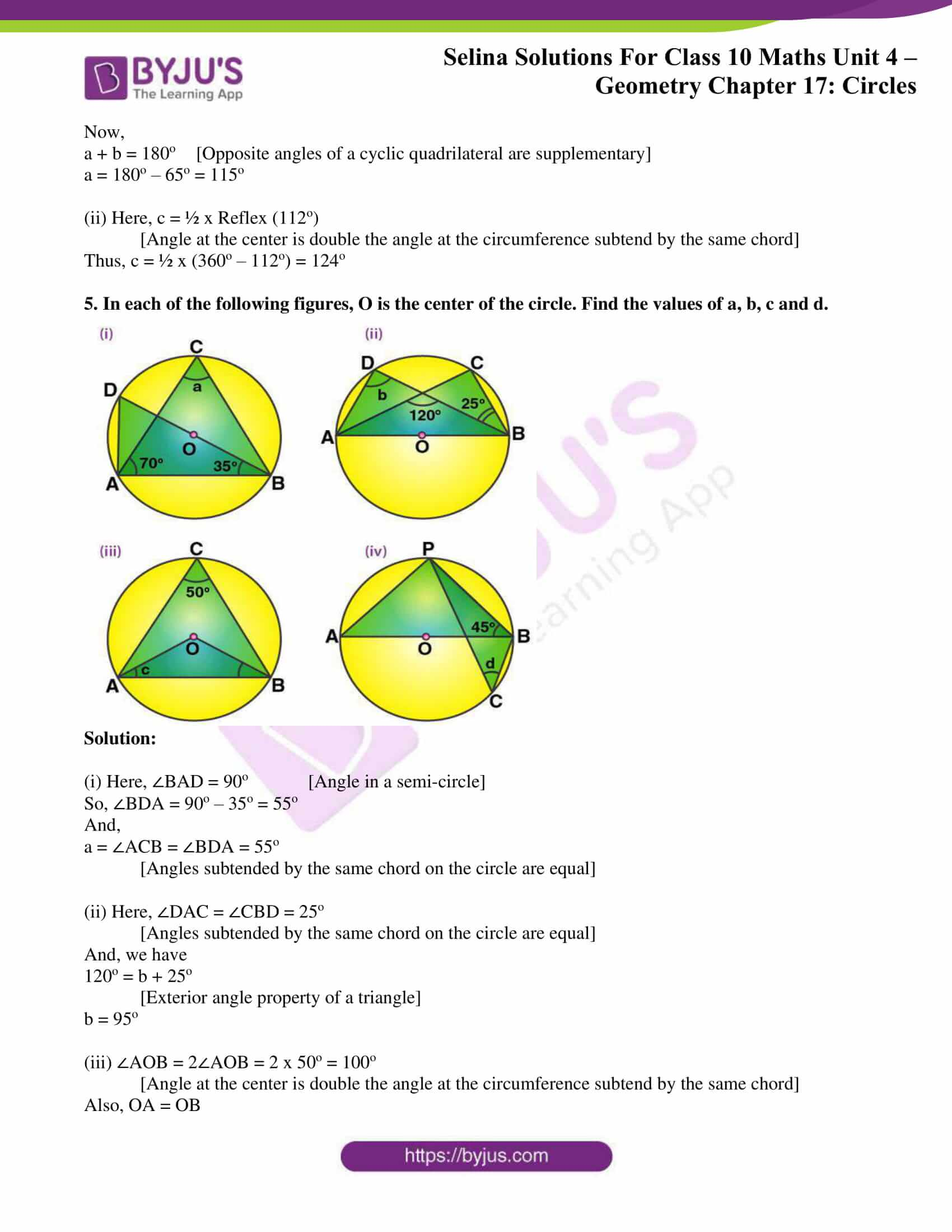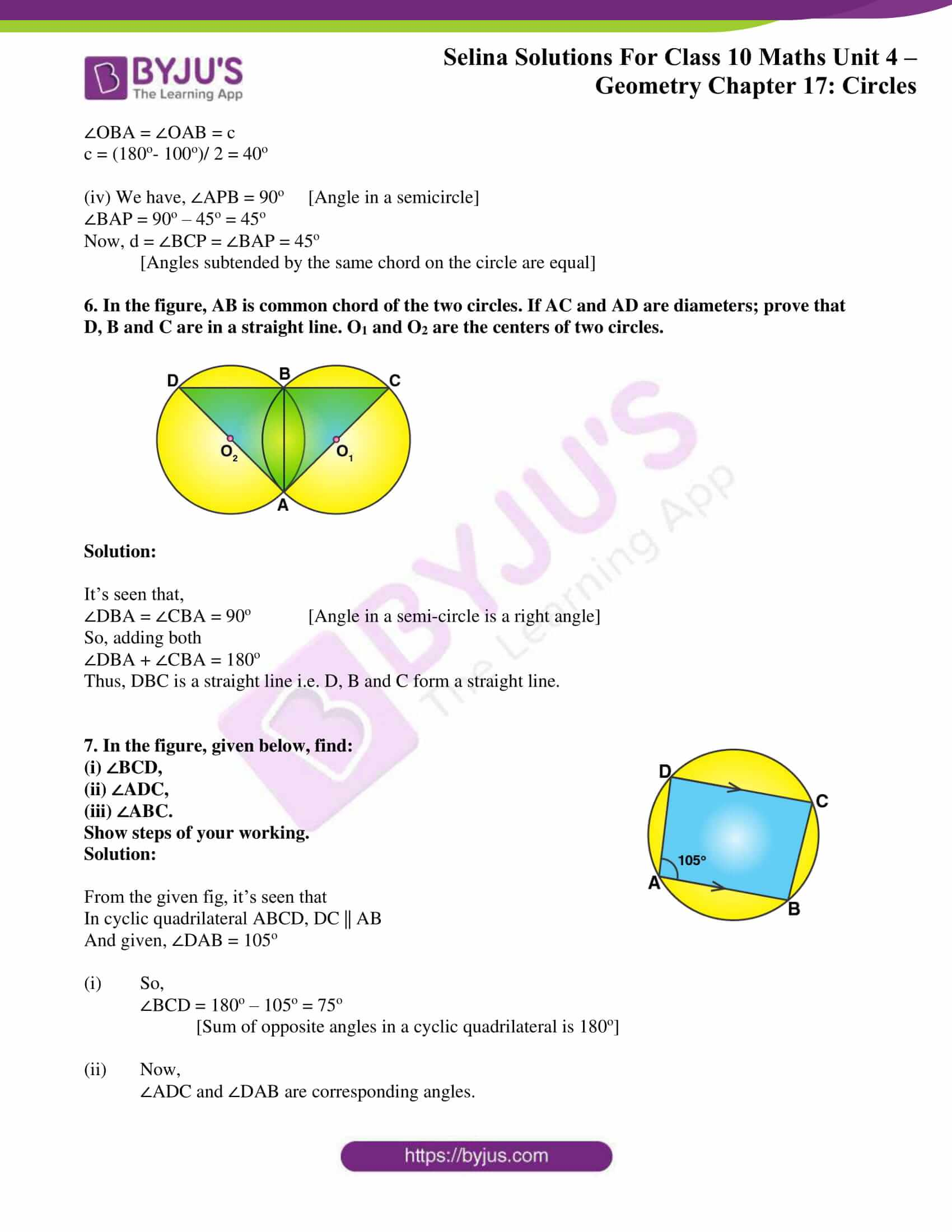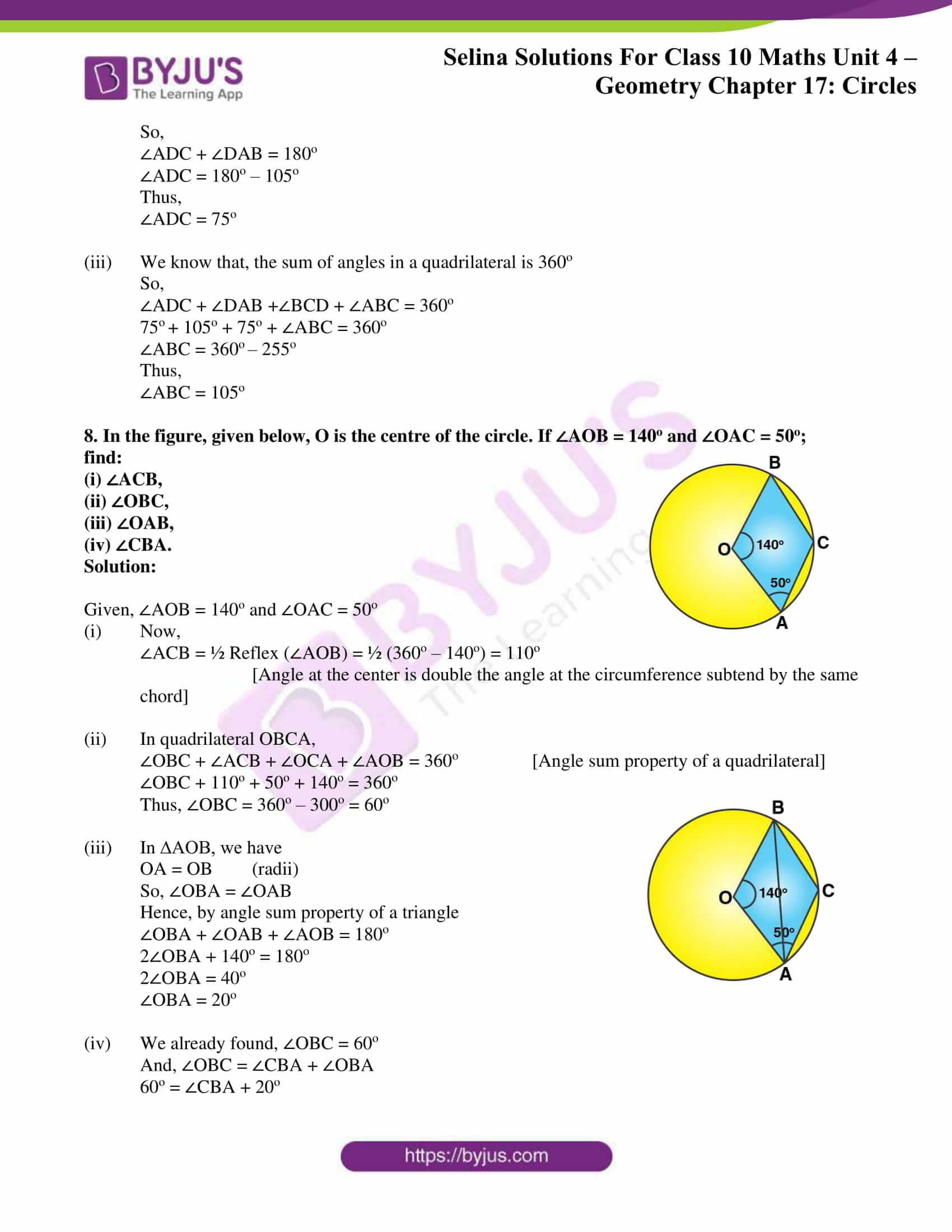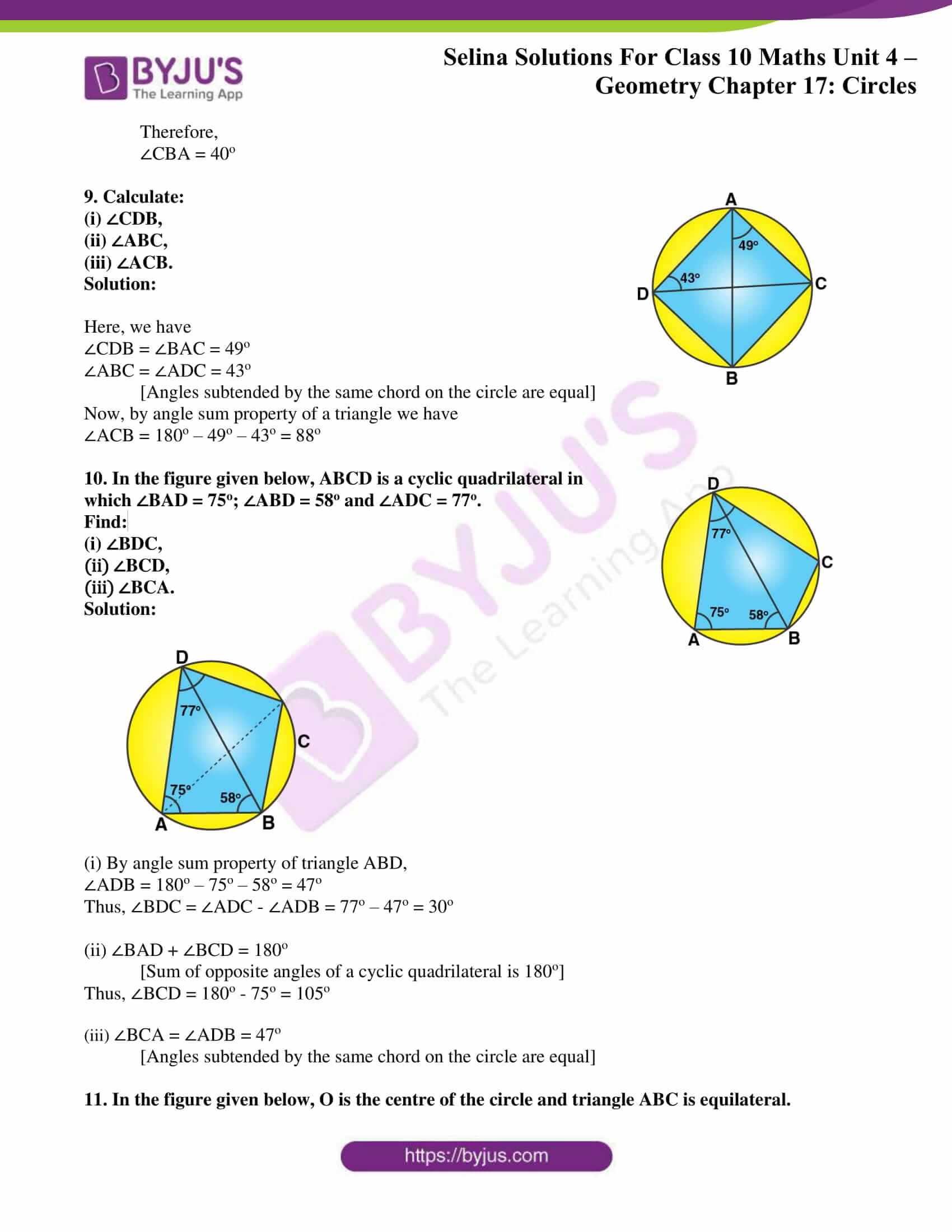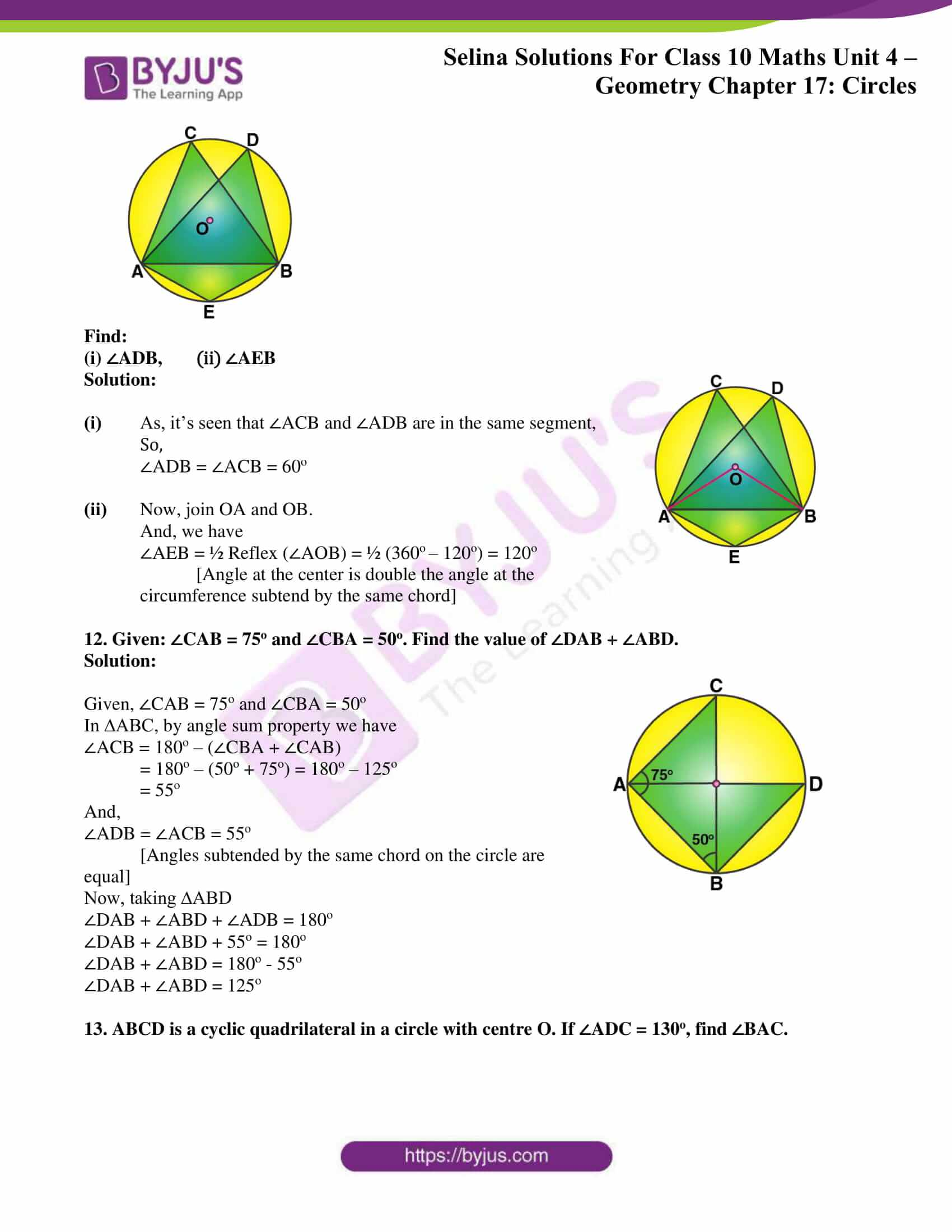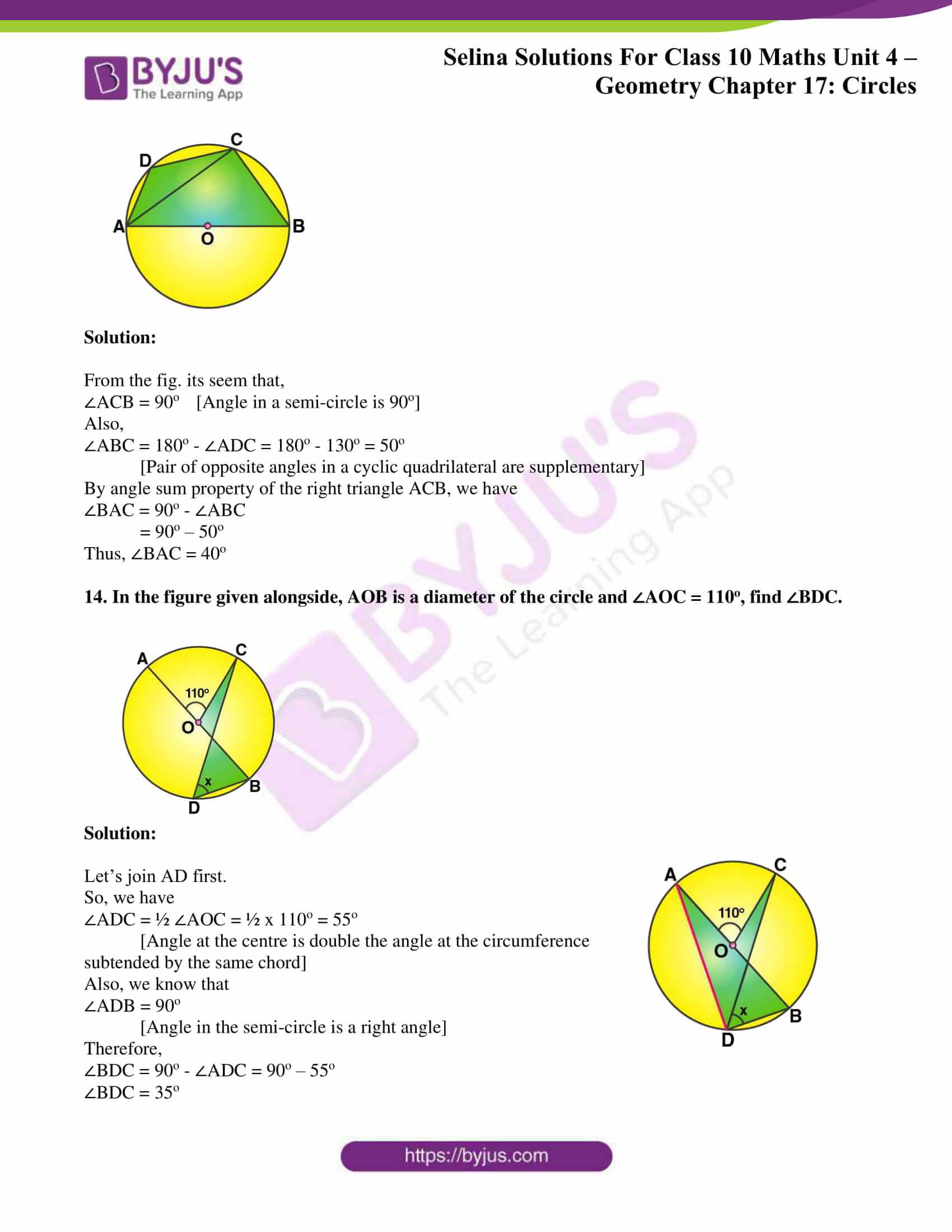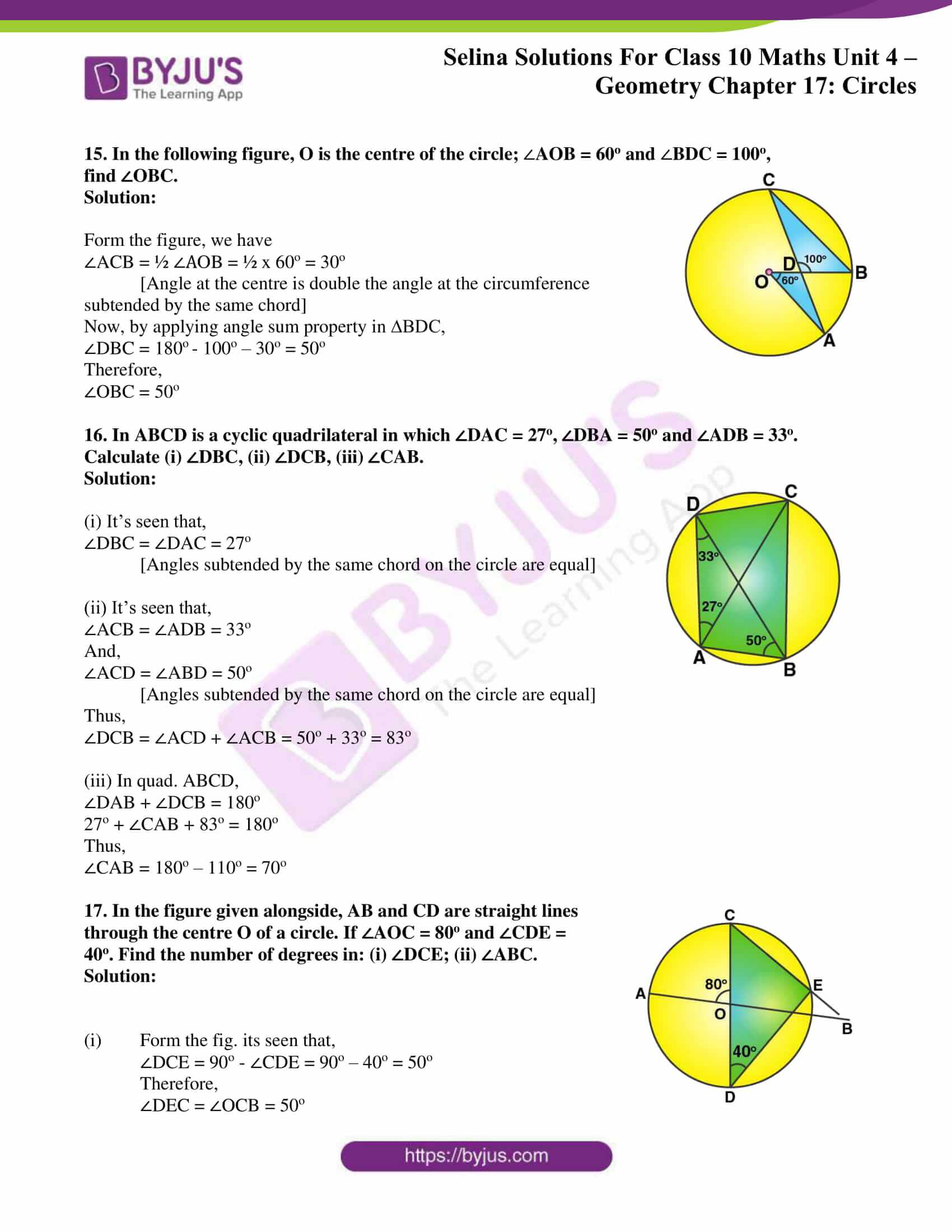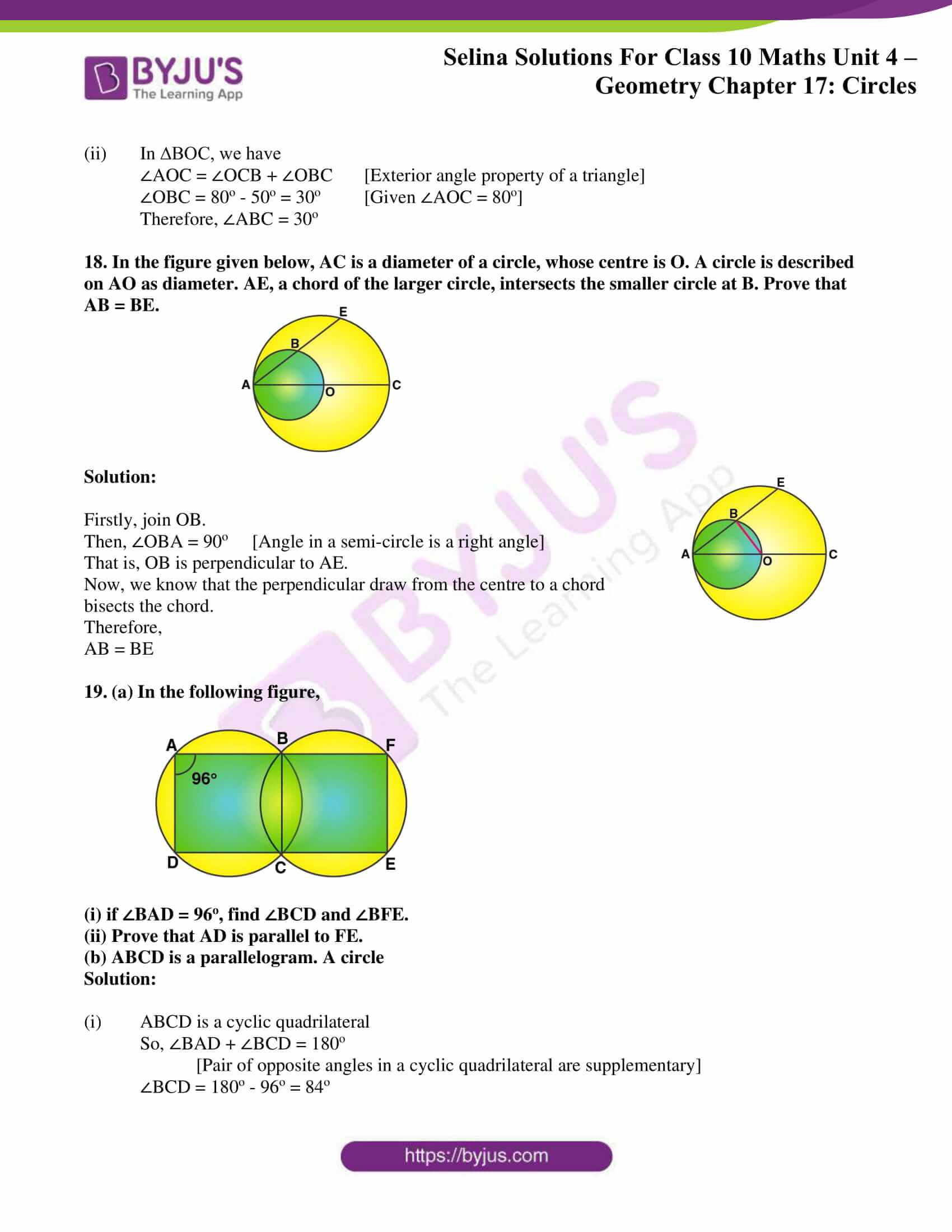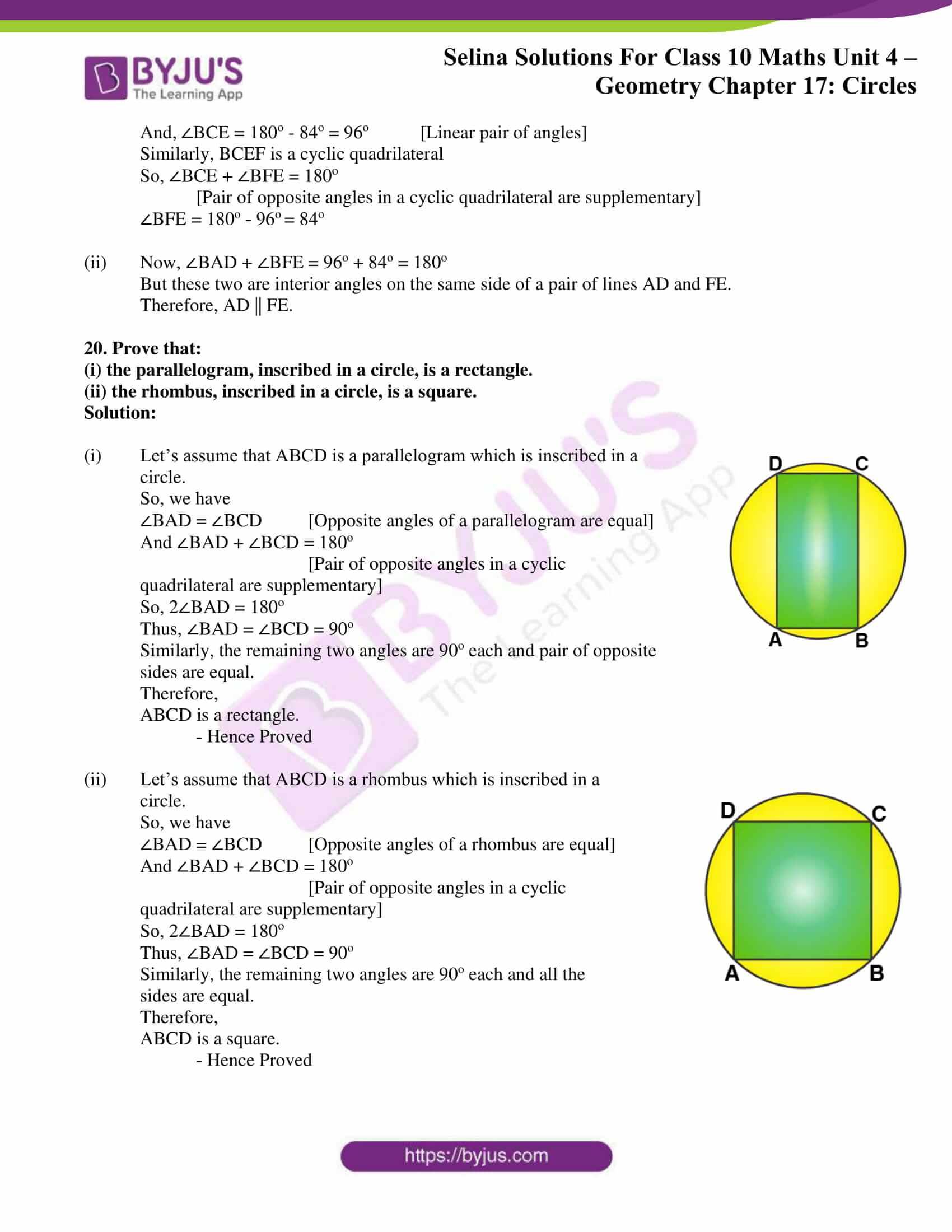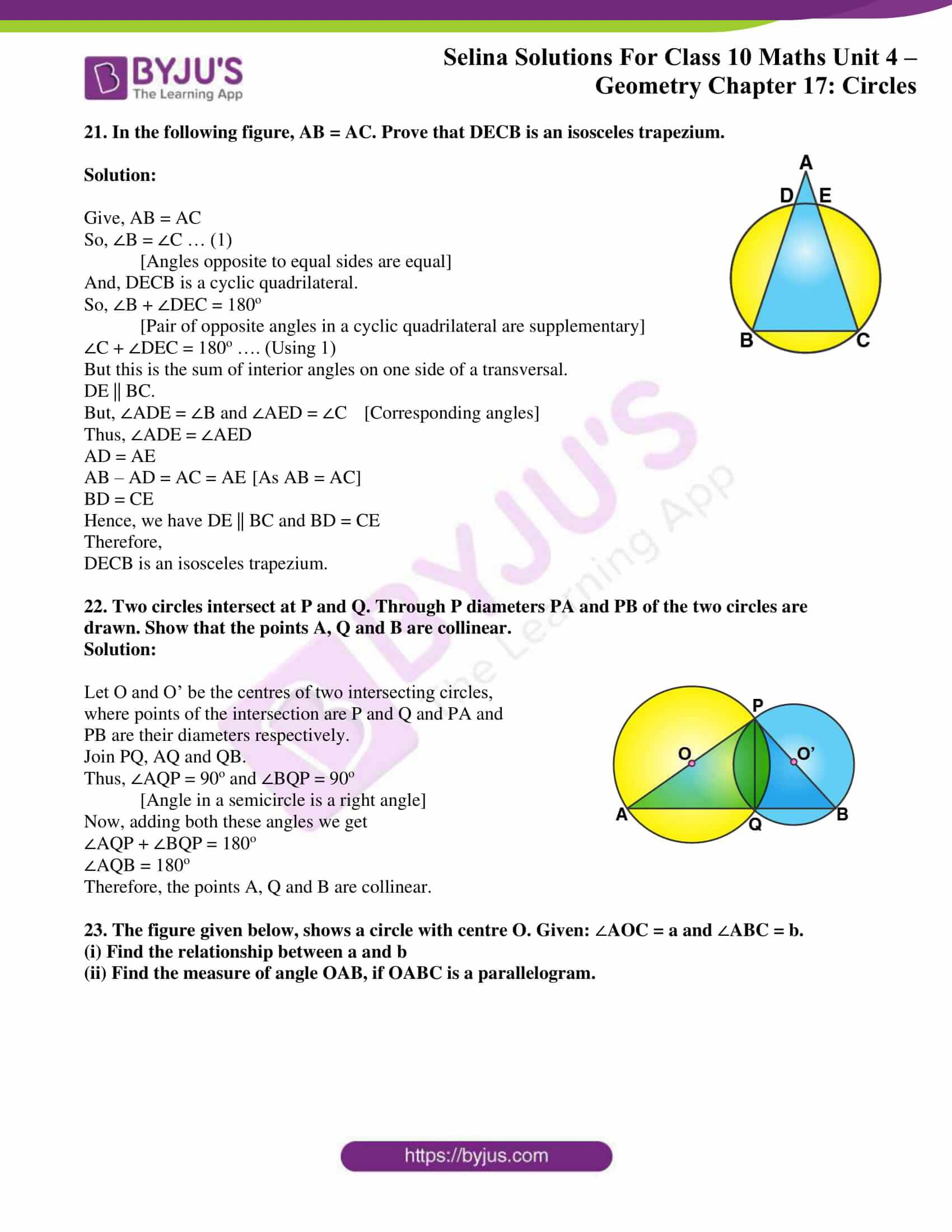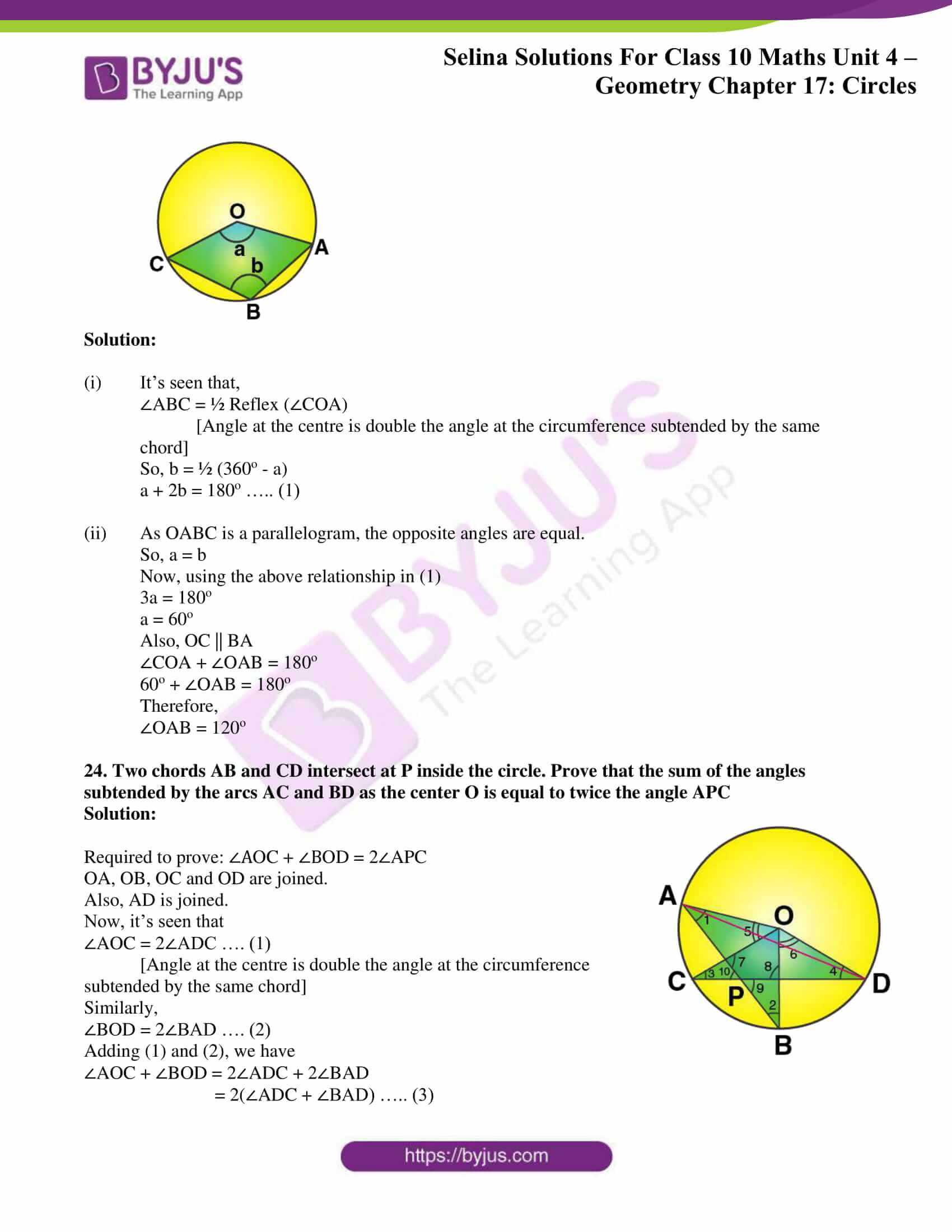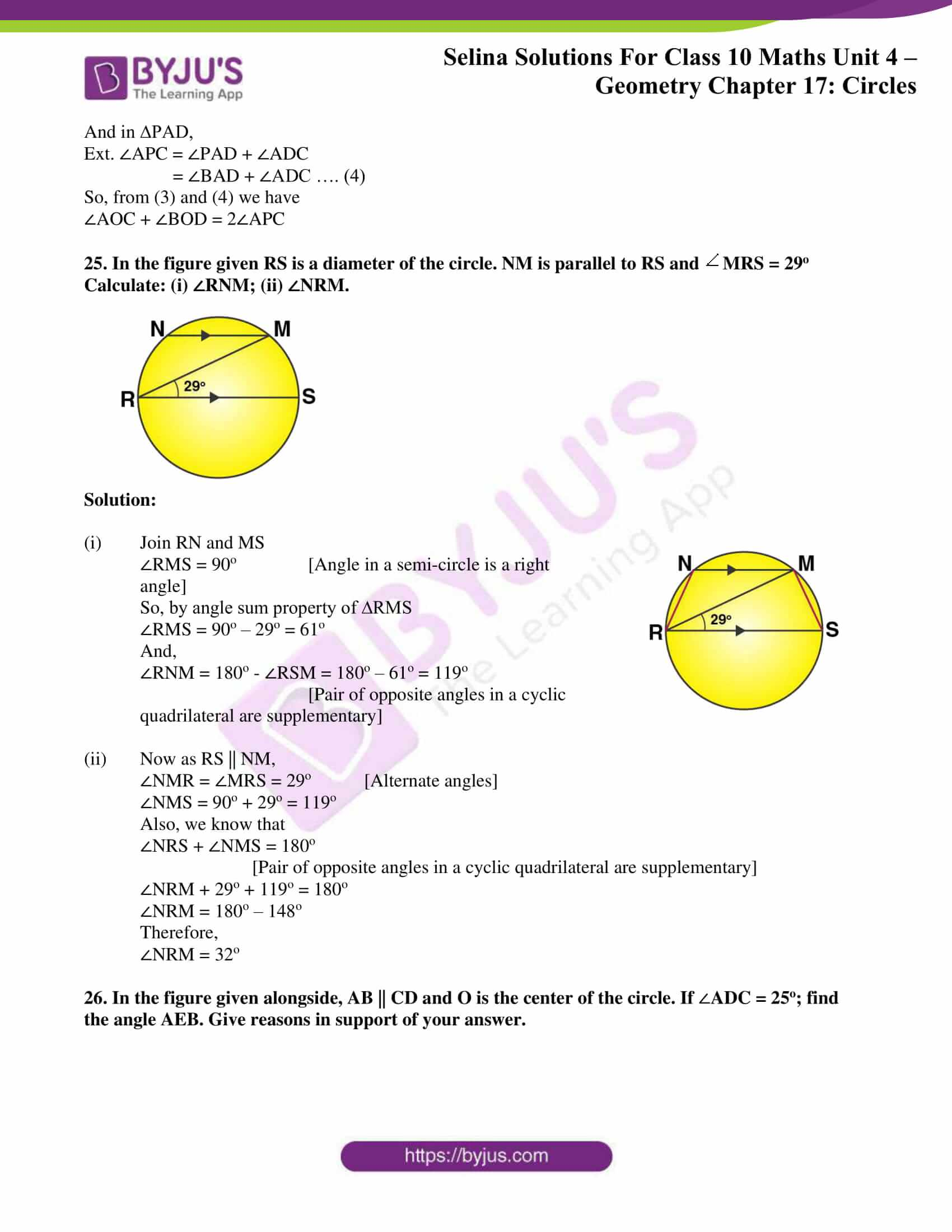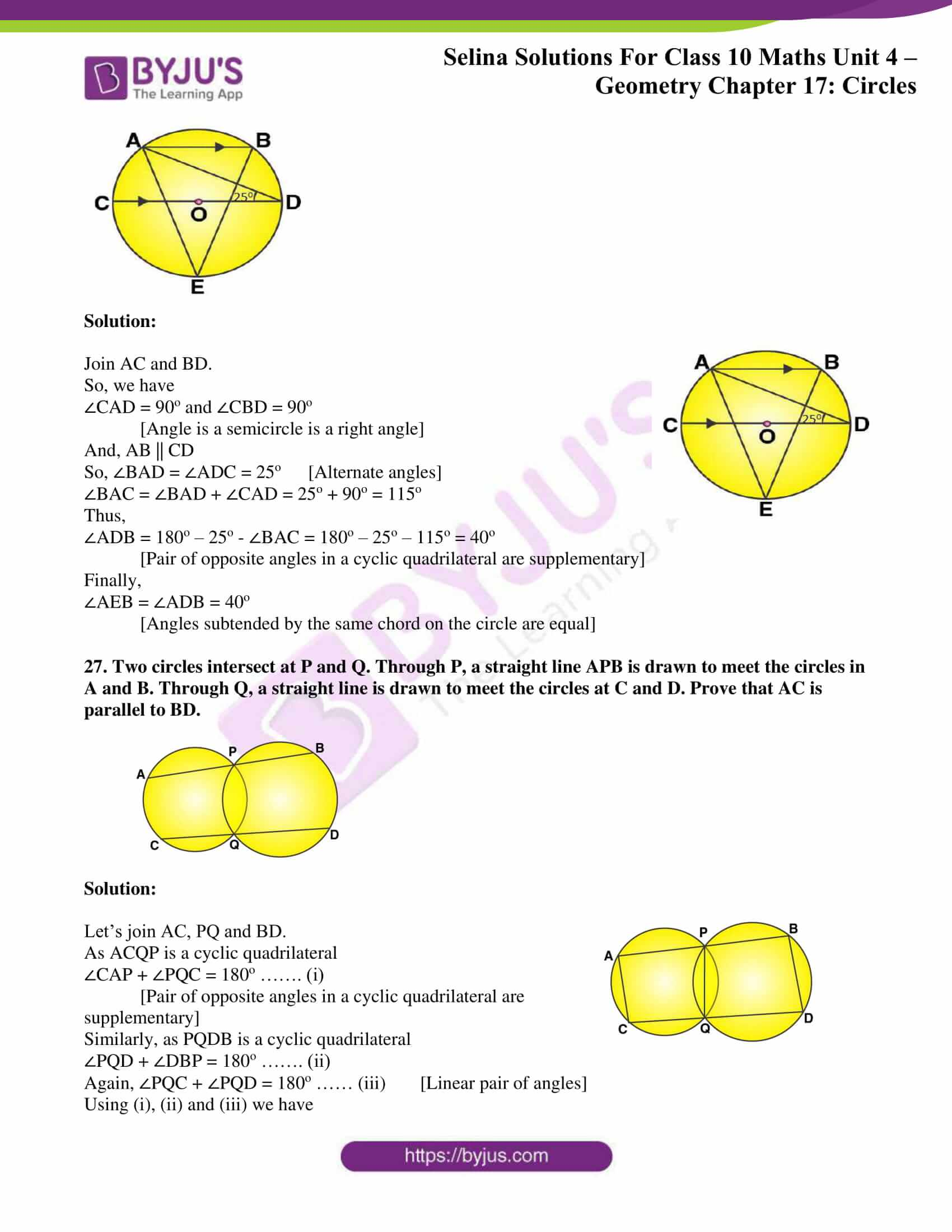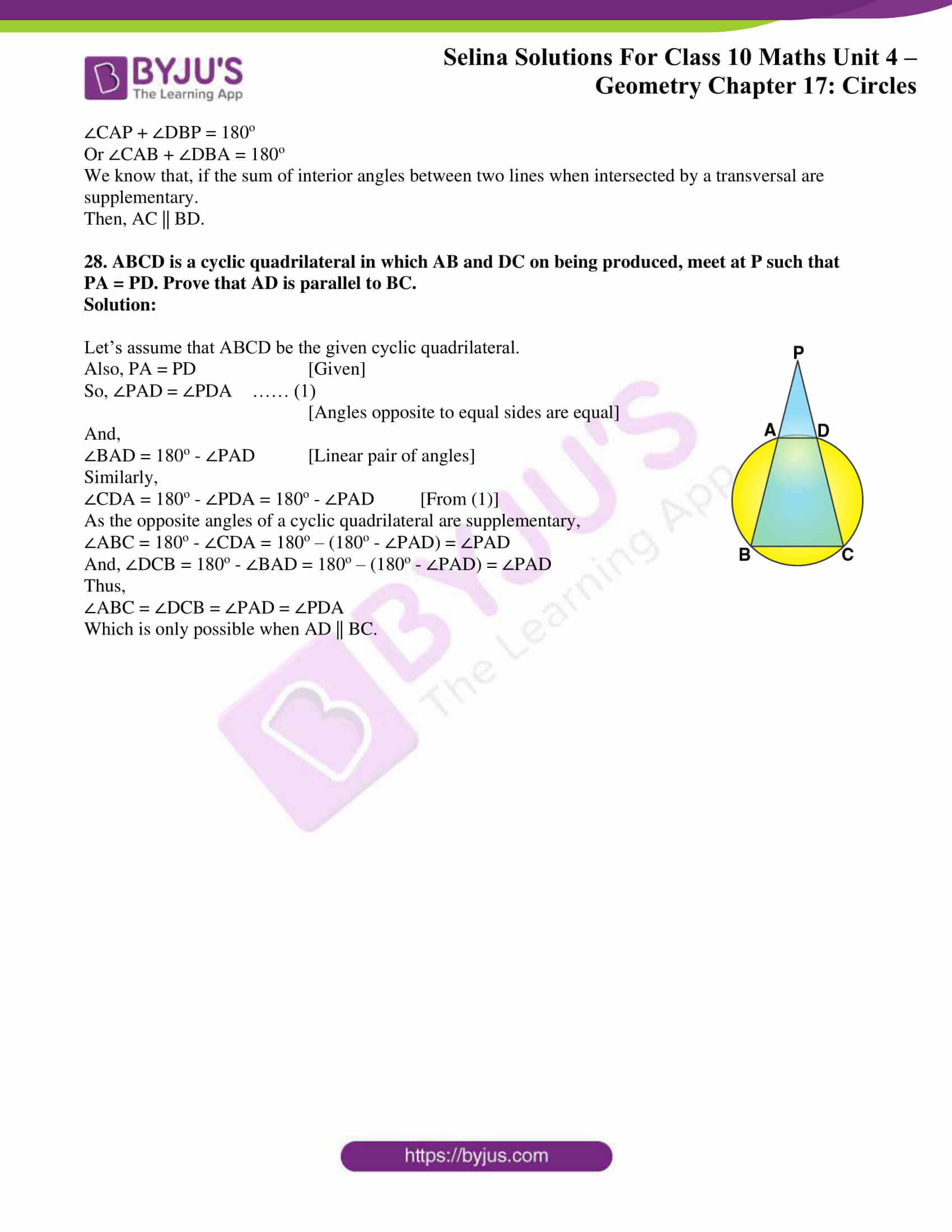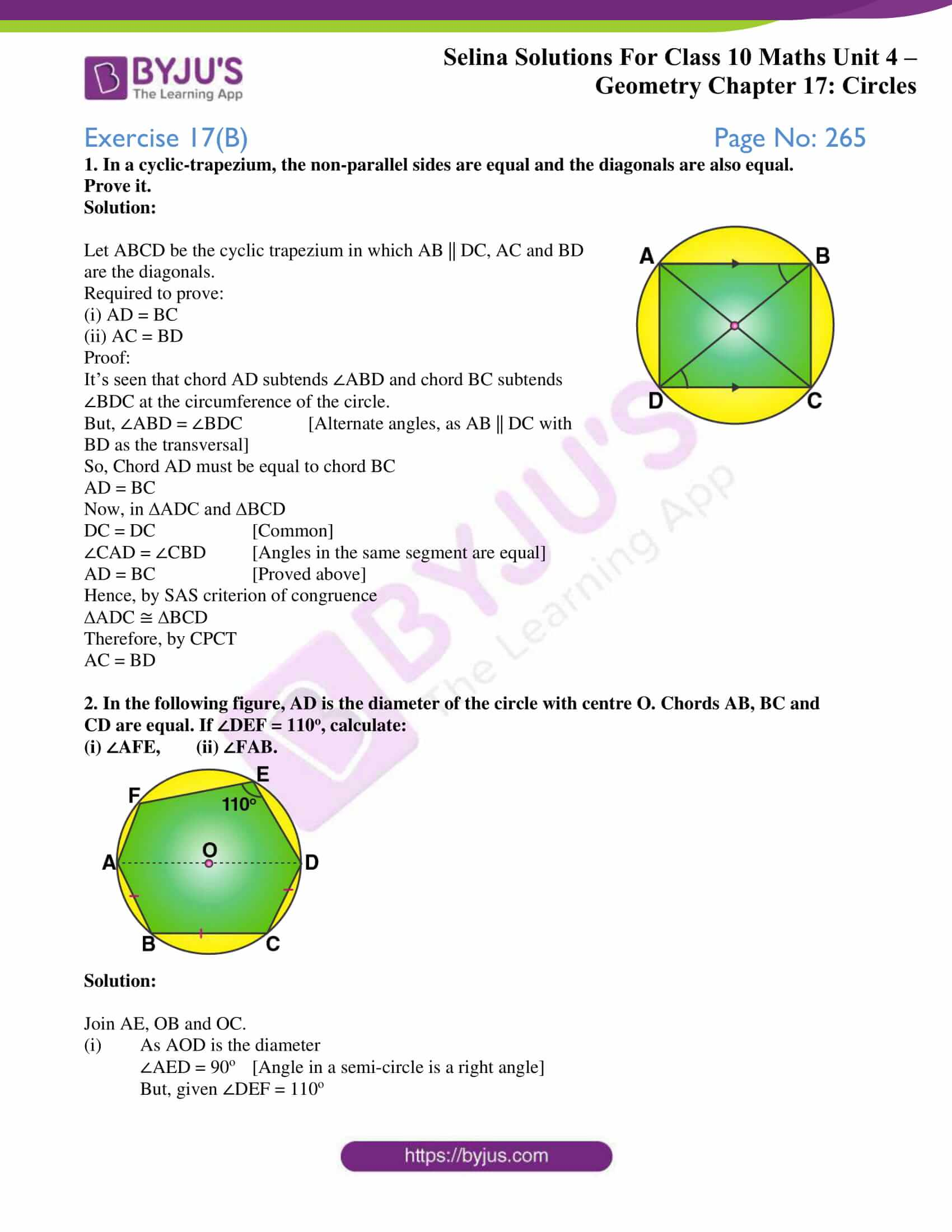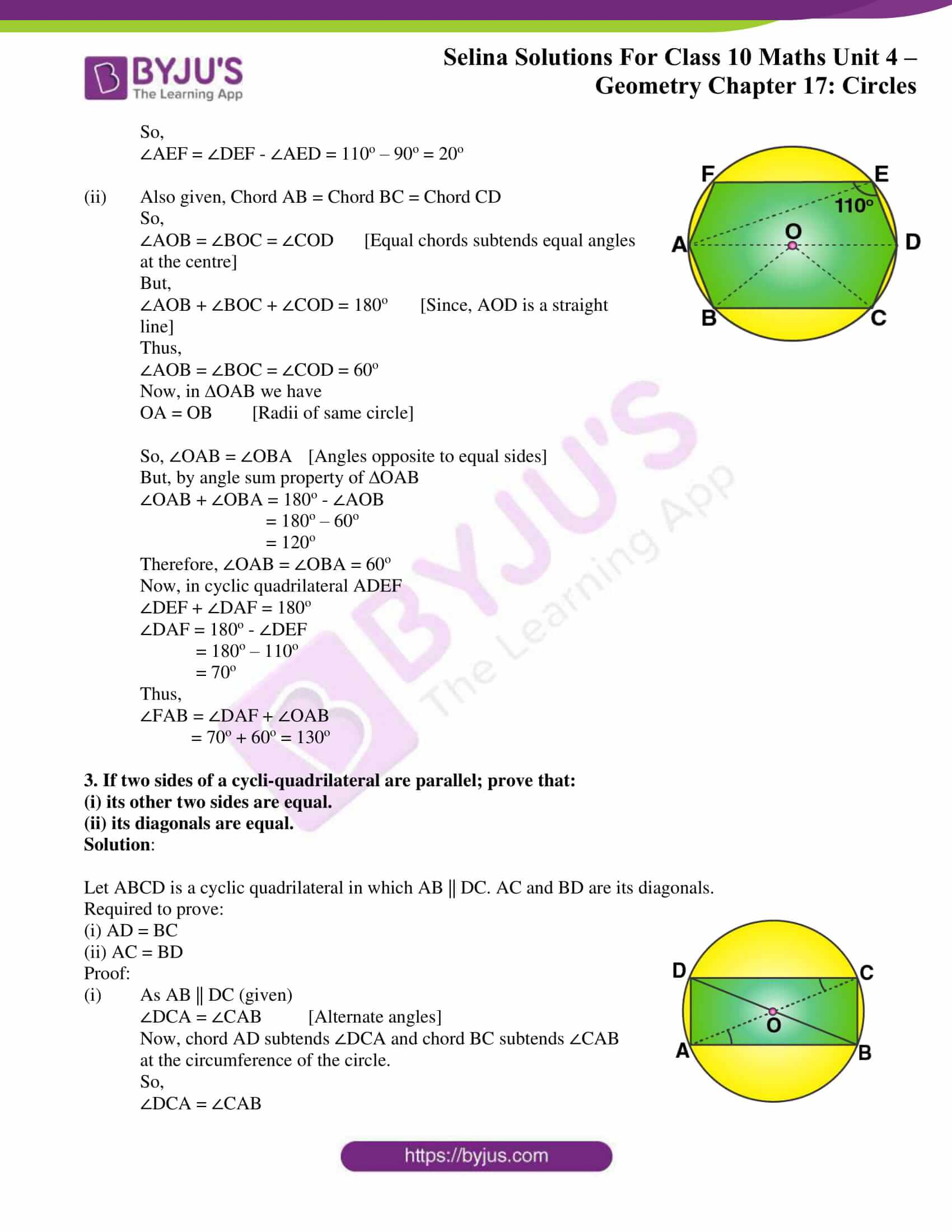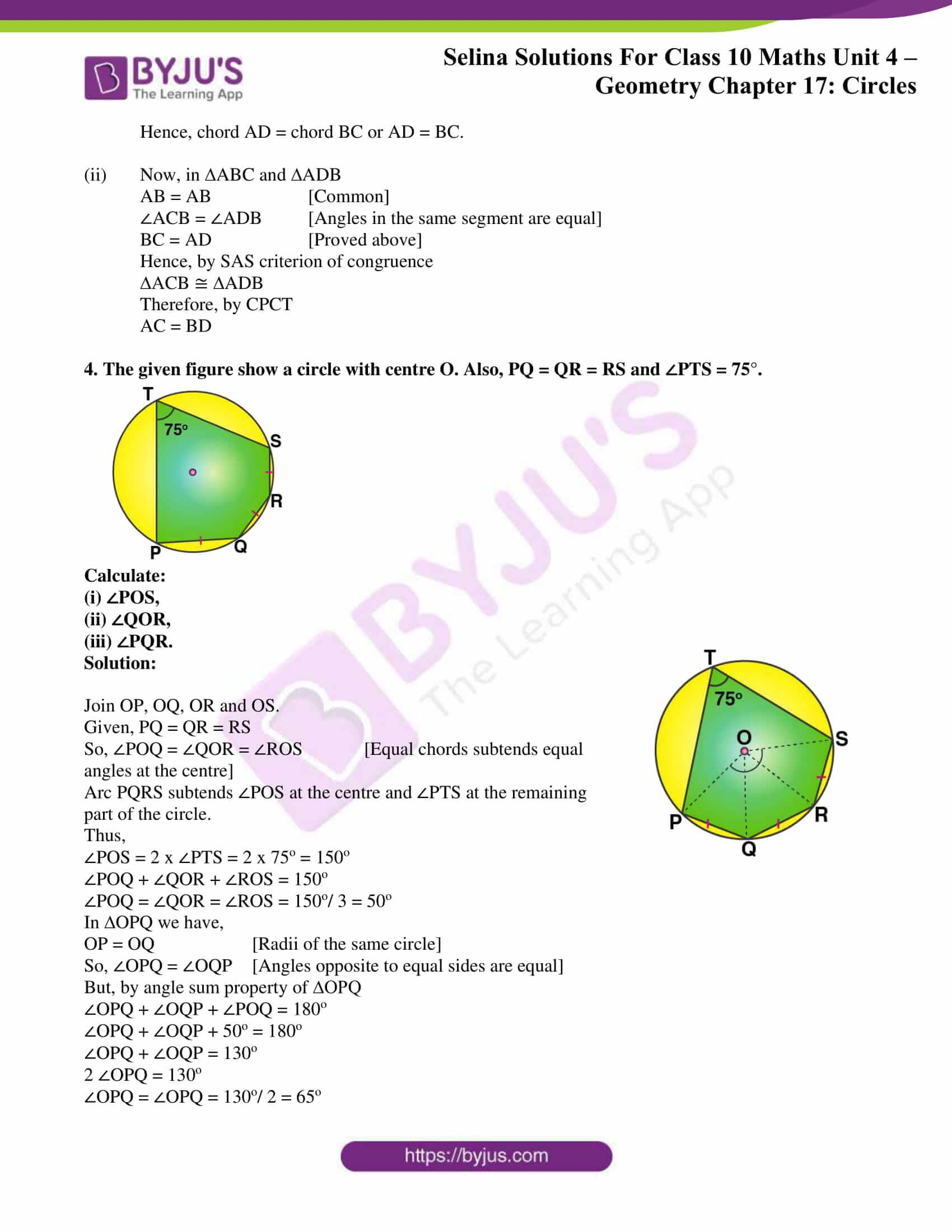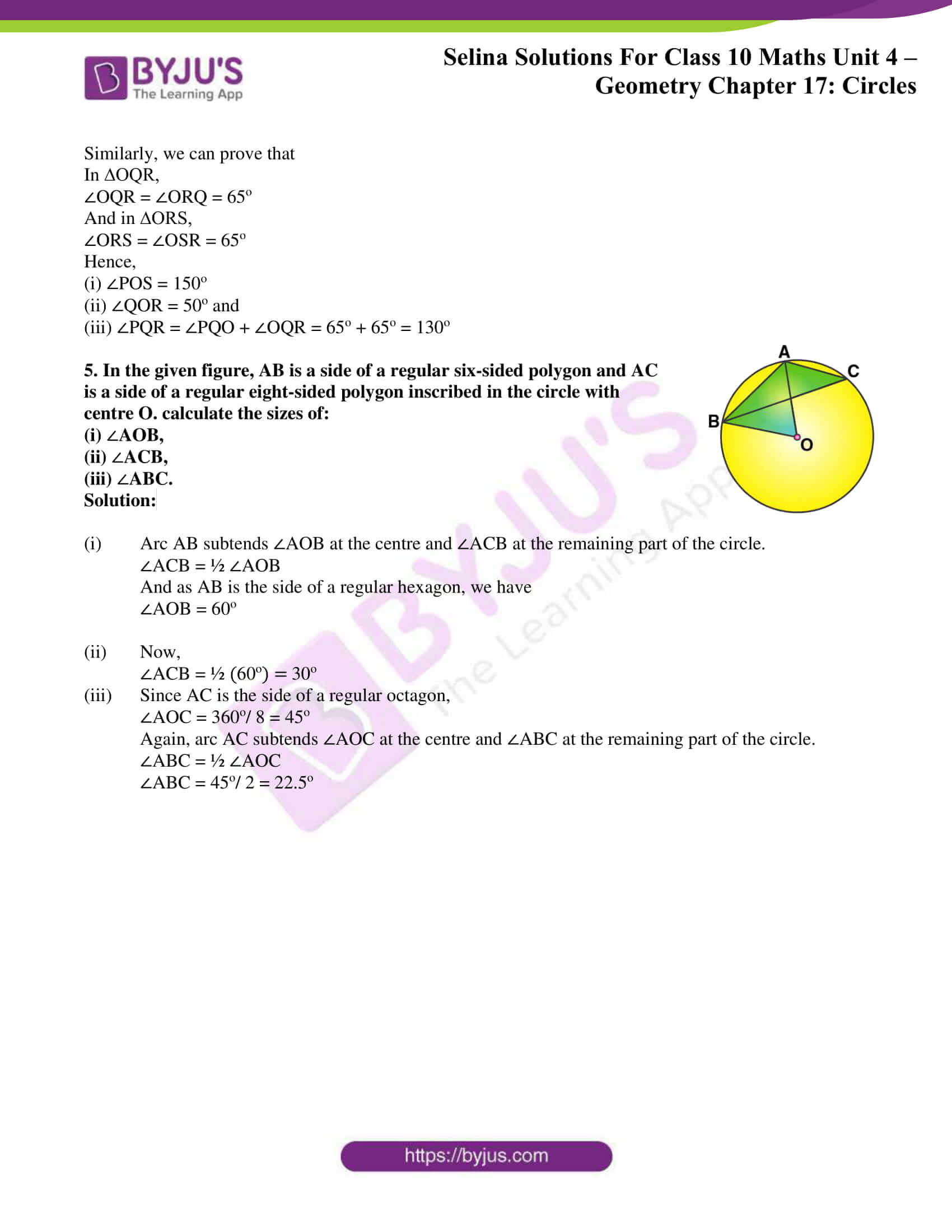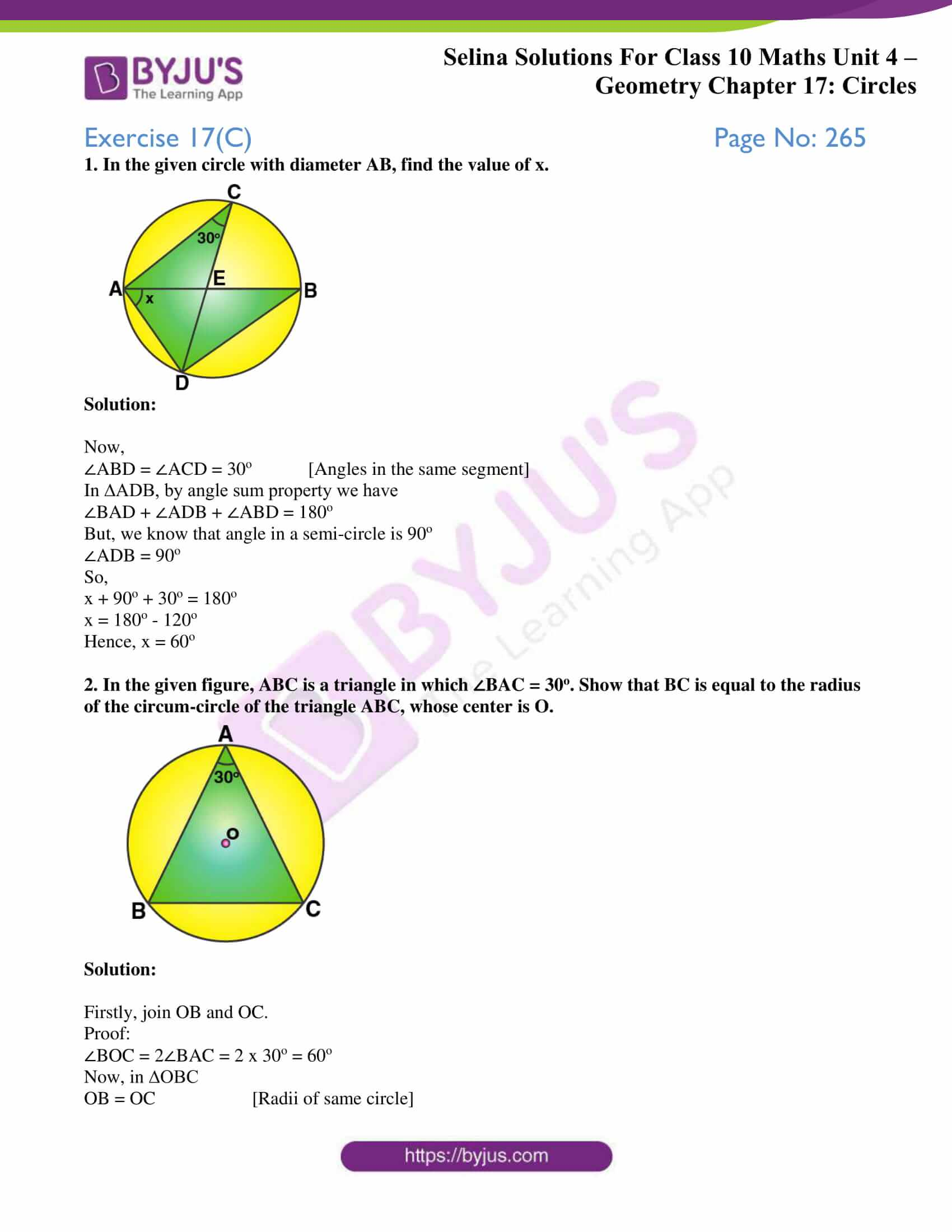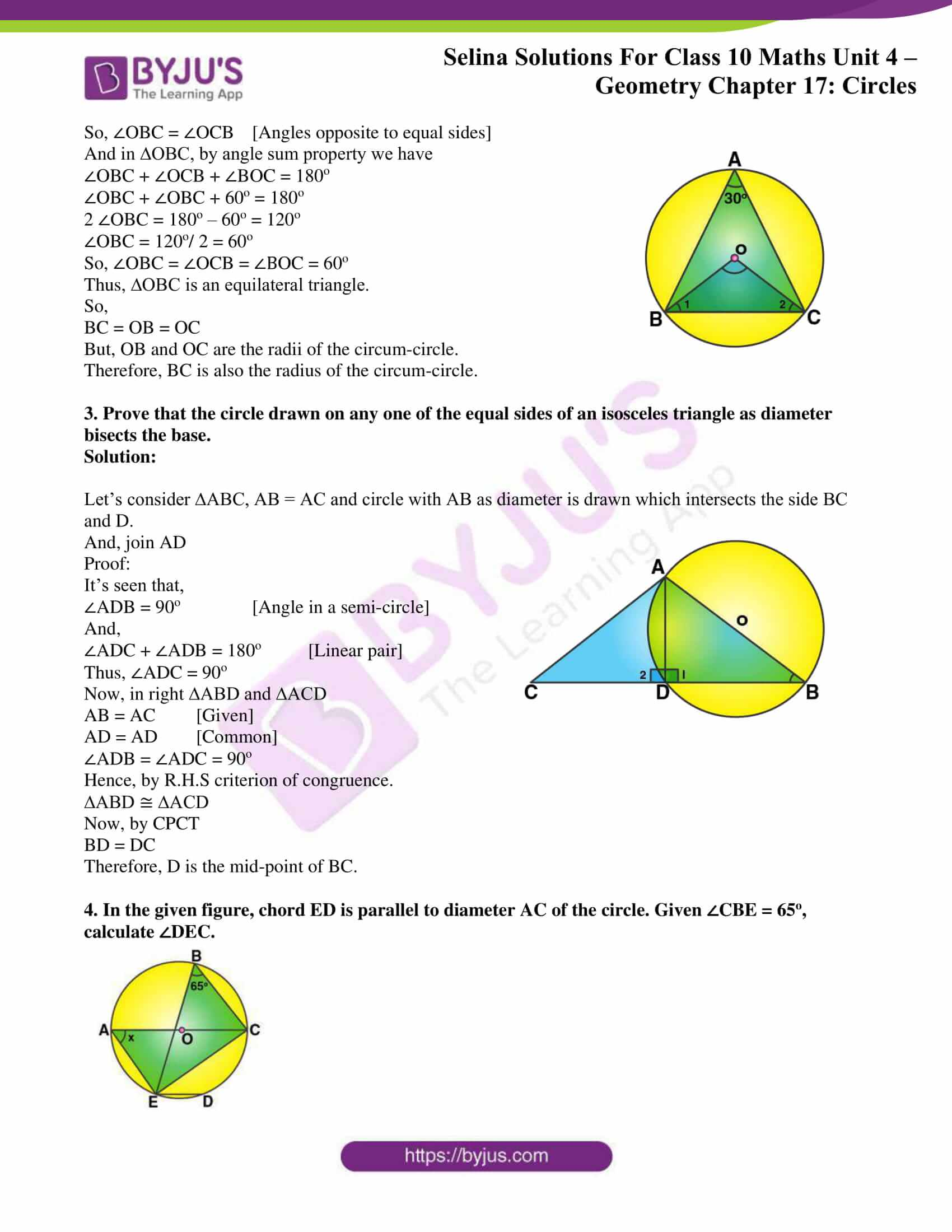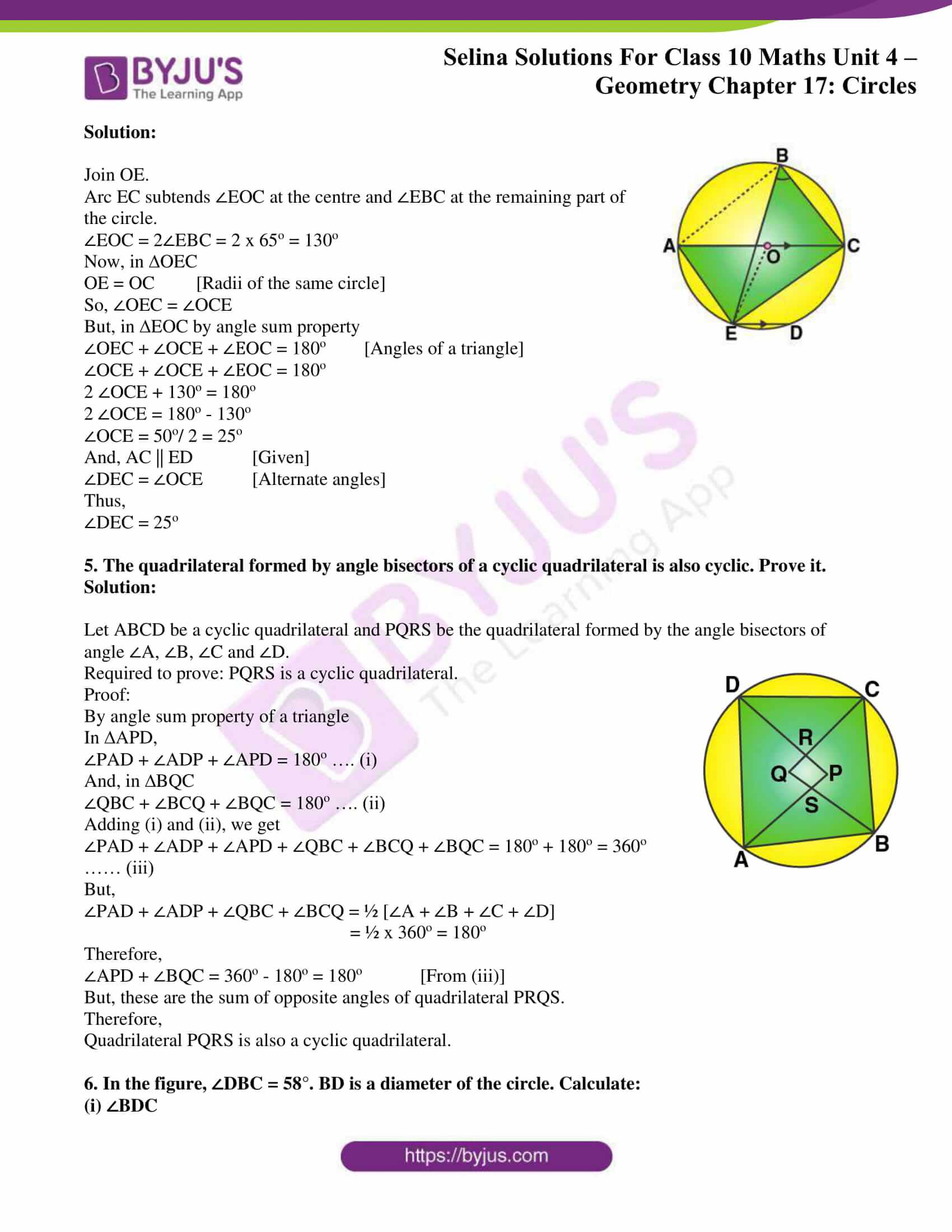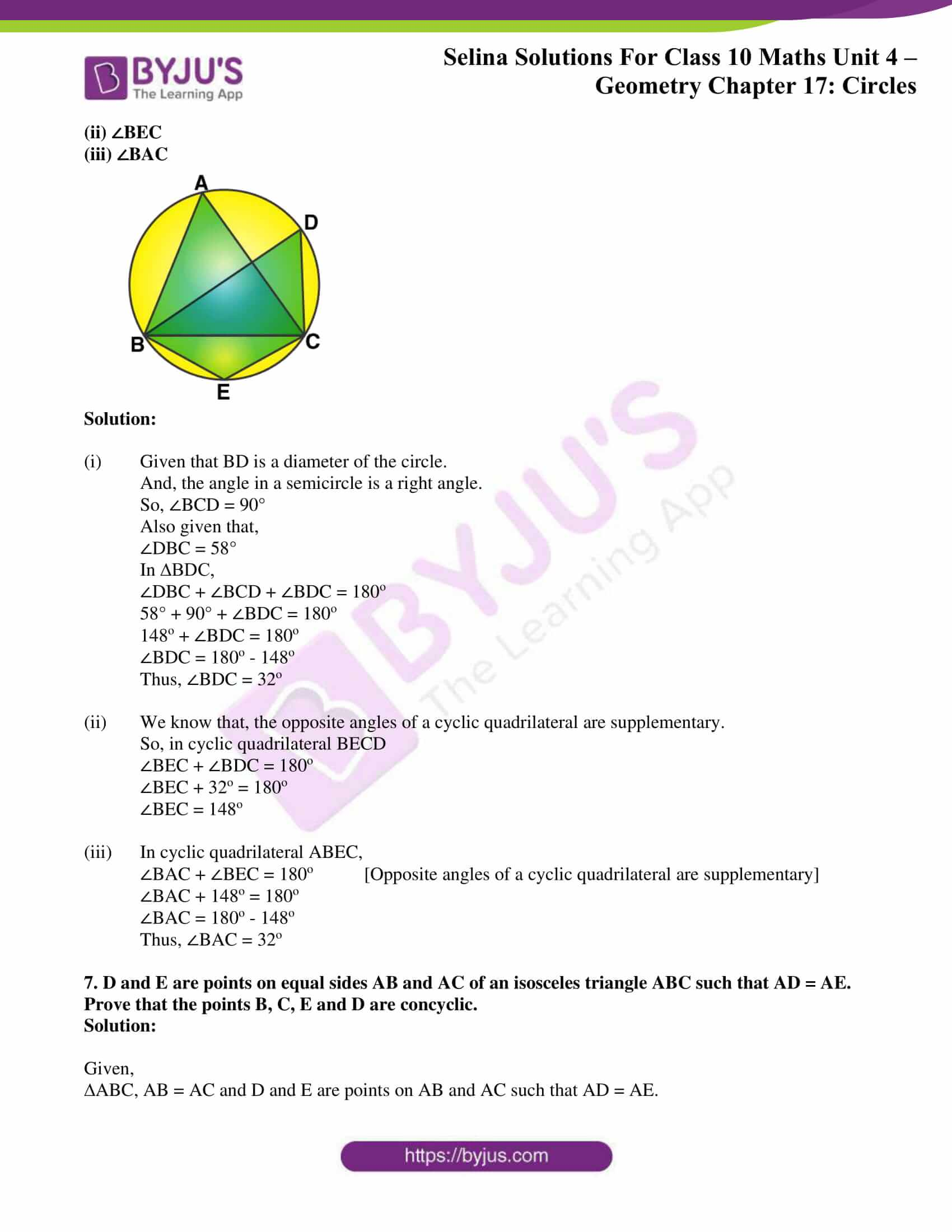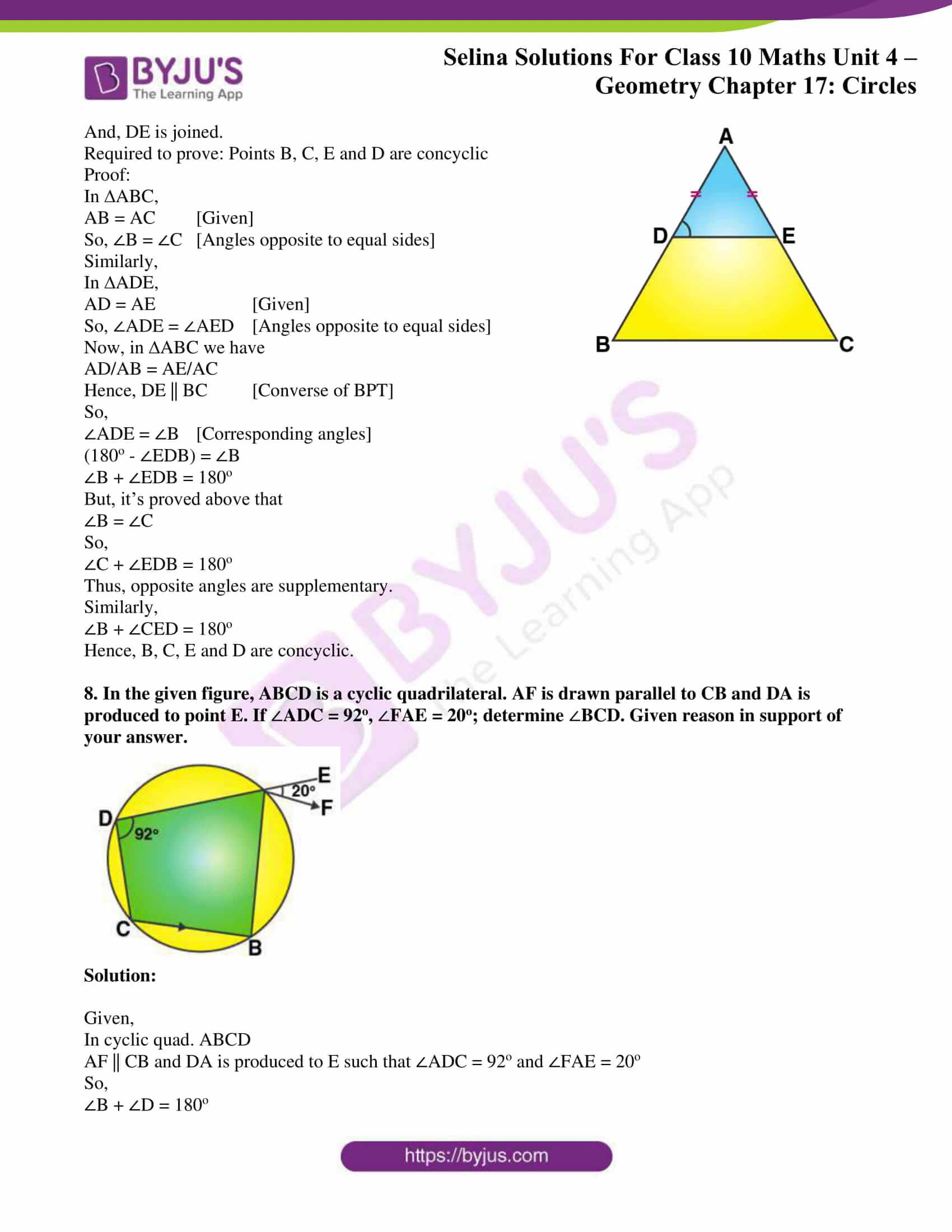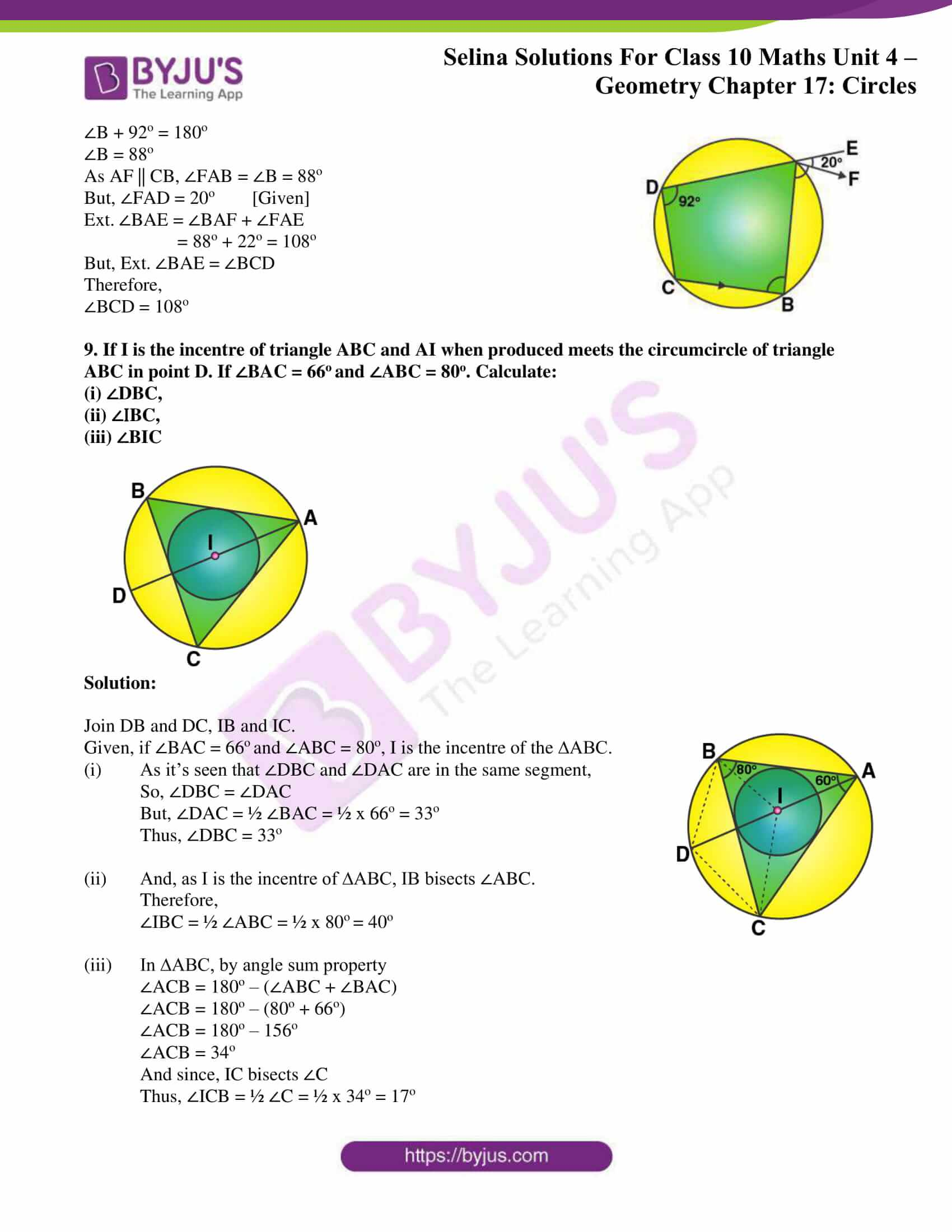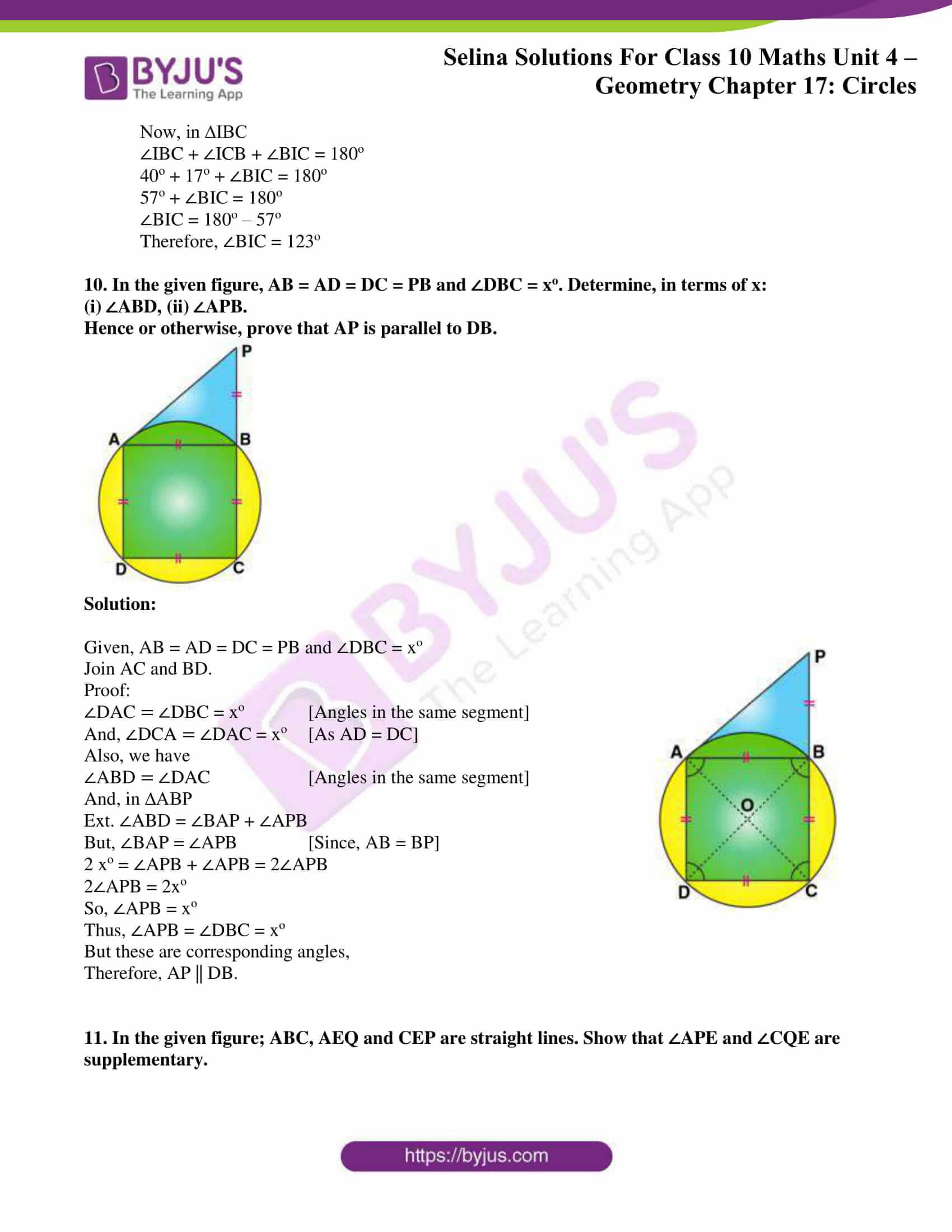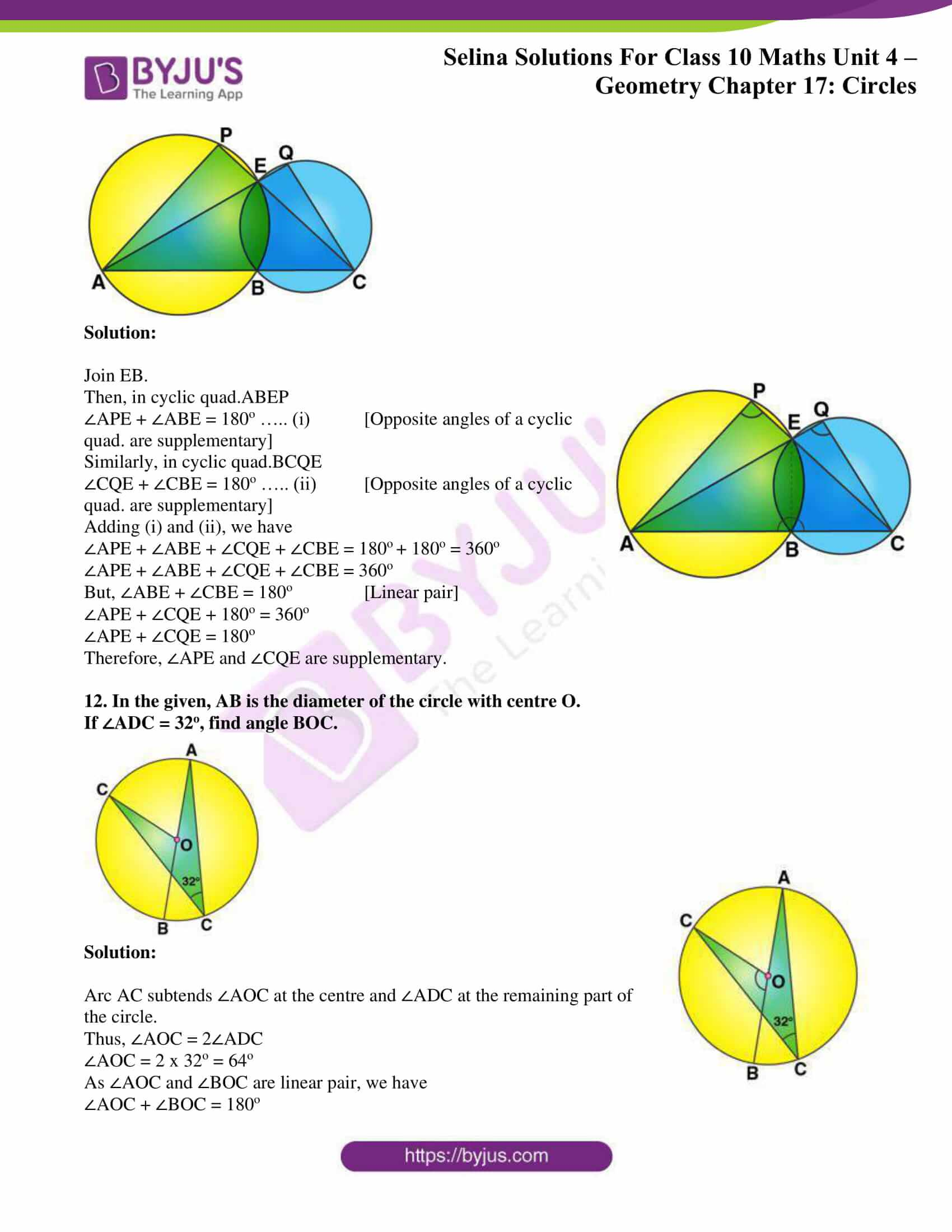### Exercises of Concise Selina Solutions Class 10 Maths Chapter 17 Circles

Exercise 17(A) Solutions

Exercise 17(B) Solutions

Exercise 17(C) Solutions

## Access Selina Solutions Concise Maths Class 10 Chapter 17 Circles

Exercise 17(A) Page No: 257

1. In the given figure, O is the center of the circle. ∠OAB and ∠OCB are 30o and 40o respectively. Find ∠AOC Show your steps of working.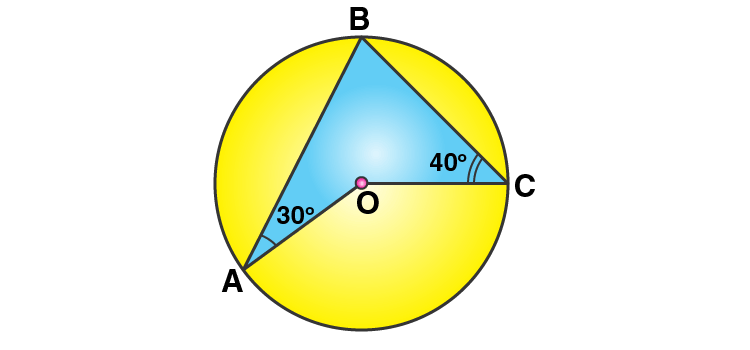Solution: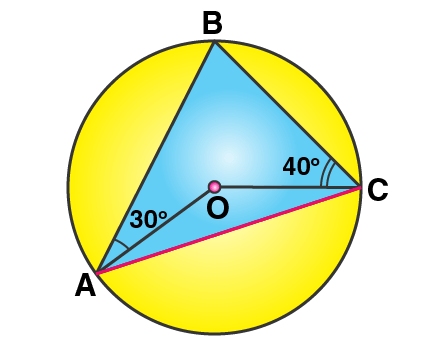Firstly, let’s join AC.

And, let ∠OAC = ∠OCA = x [Angles opposite to equal sides are equal]

So, ∠AOC = 180o – 2x

Also,

∠BAC = 30o + x

∠BCA = 40o + x

Now, in ∆ABC

∠ABC = 180o – ∠BAC – ∠BCA [Angles sum property of a triangle]

= 180o – (30o + x) – (40o + x)

= 110o – 2x

And, ∠AOC = 2∠ABC

[Angle at the center is double the angle at the circumference subtend by the same chord]

180o – 2x = 2(110o – 2x)

2x = 40o

x = 20o

Thus, ∠AOC = 180o – 2×20o = 140o

2. In the given figure, ∠BAD = 65°, ∠ABD = 70°, ∠BDC = 45°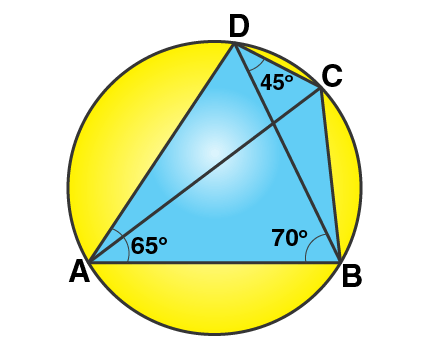(i) Prove that AC is a diameter of the circle.

(ii) Find ∠ACB.

Solution:

(i) In ∆ABD,

∠DAB + ∠ABD + ∠ADB = 180o

65o + 70o + ∠ADB = 180o

∠ADB = 180o – 135o = 45o

Now,

As ∠ADC is the angle of semi-circle for AC as the diameter of the circle.

(ii) ∠ACB = ∠ADB [Angles in the same segment of a circle]

Hence, ∠ACB = 45o

3. Given O is the centre of the circle and ∠AOB = 70o.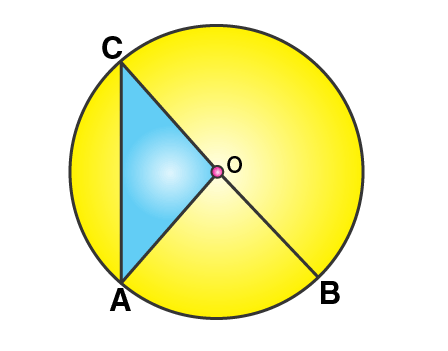Calculate the value of:

(i) ∠OCA,

(ii) ∠OAC.

Solution:

Here, ∠AOB = 2∠ACB

[Angle at the center is double the angle at the circumference subtend by the same chord]

∠ACB = 70o/ 2 = 35o

Now, OC = OA [Radii of same circle]

Thus,

∠OCA = ∠OAC = 35o

4. In each of the following figures, O is the centre of the circle. Find the values of a, b and c.

Solution:

(i)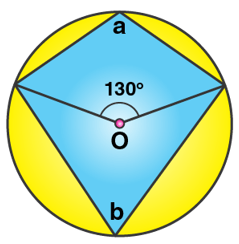(ii)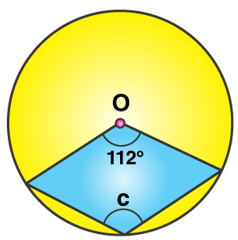(i) Here, b = ½ x 130o

[Angle at the center is double the angle at the circumference subtend by the same chord]

Thus, b = 65o

Now,

a + b = 180o [Opposite angles of a cyclic quadrilateral are supplementary]

a = 180o – 65o = 115o

(ii) Here, c = ½ x Reflex (112o)

[Angle at the center is double the angle at the circumference subtend by the same chord]

Thus, c = ½ x (360o – 112o) = 124o

5. In each of the following figures, O is the center of the circle. Find the values of a, b, c and d.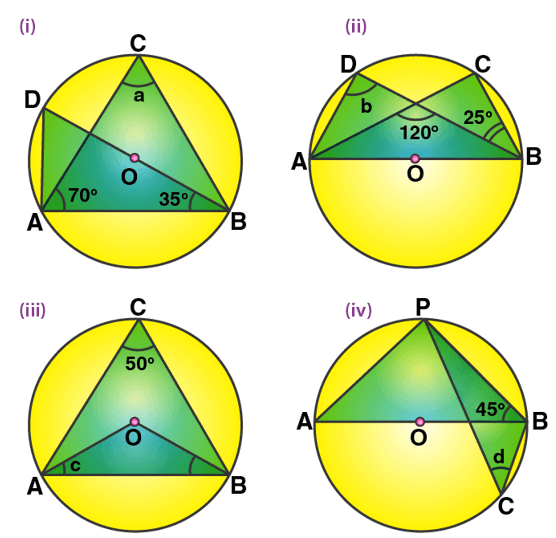Solution:

(i) Here, ∠BAD = 90o [Angle in a semi-circle]

So, ∠BDA = 90o – 35o = 55o

And,

a = ∠ACB = ∠BDA = 55o

[Angles subtended by the same chord on the circle are equal]

(ii) Here, ∠DAC = ∠CBD = 25o

[Angles subtended by the same chord on the circle are equal]

And, we have

120o = b + 25o

[Exterior angle property of a triangle]

b = 95o

(iii) ∠AOB = 2∠AOB = 2 x 50o = 100o

[Angle at the center is double the angle at the circumference subtend by the same chord]

Also, OA = OB

∠OBA = ∠OAB = c

c = (180o– 100o)/ 2 = 40o

(iv) We have, ∠APB = 90o [Angle in a semicircle]

∠BAP = 90o – 45o = 45o

Now, d = ∠BCP = ∠BAP = 45o

[Angles subtended by the same chord on the circle are equal]

6. In the figure, AB is common chord of the two circles. If AC and AD are diameters; prove that D, B and C are in a straight line. O1 and O2 are the centers of two circles.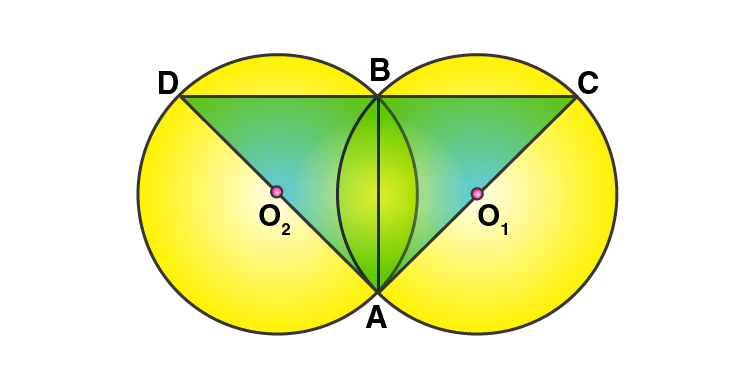Solution:

It’s seen that,

∠DBA = ∠CBA = 90o [Angle in a semi-circle is a right angle]

∠DBA + ∠CBA = 180o

Thus, DBC is a straight line i.e. D, B and C form a straight line.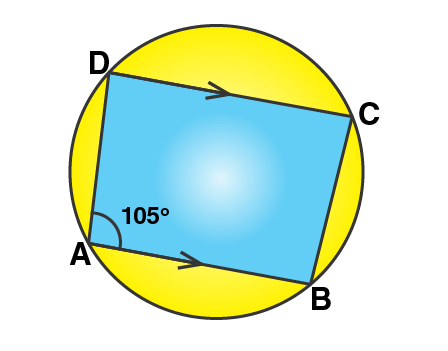7. In the figure, given below, find:

(i) ∠BCD,

(iii) ∠ABC.

Solution:

From the given fig, it’s seen that

In cyclic quadrilateral ABCD, DC || AB

And given, ∠DAB = 105o

(i) So,

∠BCD = 180o – 105o = 75o

[Sum of opposite angles in a cyclic quadrilateral is 180o]

(ii) Now,

∠ADC and ∠DAB are corresponding angles.

So,

Thus,

(iii) We know that, the sum of angles in a quadrilateral is 360o

So,

∠ADC + ∠DAB +∠BCD + ∠ABC = 360o

75o + 105o + 75o + ∠ABC = 360o

∠ABC = 360o – 255o

Thus,

∠ABC = 105o

8. In the figure, given below, O is the centre of the circle. If ∠AOB = 140o and ∠OAC = 50o;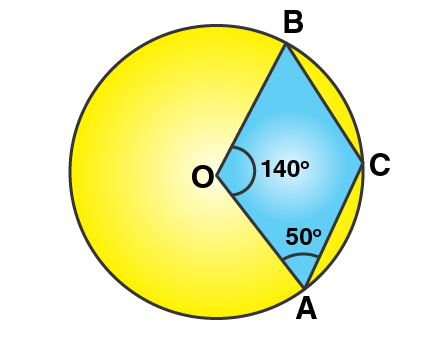find:

(i) ∠ACB,

(ii) ∠OBC,

(iii) ∠OAB,

(iv) ∠CBA.

Solution:

Given, ∠AOB = 140o and ∠OAC = 50o

(i) Now,

∠ACB = ½ Reflex (∠AOB) = ½ (360o – 140o) = 110o

[Angle at the center is double the angle at the circumference subtend by the same chord]

∠OBC + ∠ACB + ∠OCA + ∠AOB = 360o [Angle sum property of a quadrilateral]

∠OBC + 110o + 50o + 140o = 360o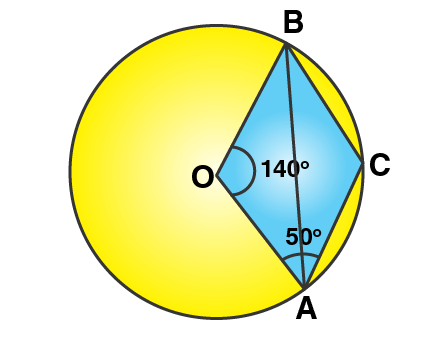Thus, ∠OBC = 360o – 300o = 60o

(iii) In ∆AOB, we have

So, ∠OBA = ∠OAB

Hence, by angle sum property of a triangle

∠OBA + ∠OAB + ∠AOB = 180o

2∠OBA + 140o = 180o

2∠OBA = 40o

∠OBA = 20o

(iv) We already found, ∠OBC = 60o

And, ∠OBC = ∠CBA + ∠OBA

60o = ∠CBA + 20o

Therefore,

∠CBA = 40o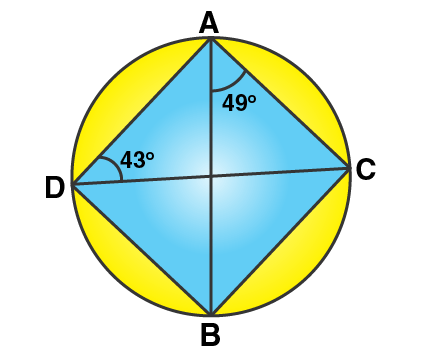9. Calculate:

(i) ∠CDB,

(ii) ∠ABC,

(iii) ∠ACB.

Solution:

Here, we have

∠CDB = ∠BAC = 49o

[Angles subtended by the same chord on the circle are equal]

Now, by angle sum property of a triangle we have

∠ACB = 180o – 49o – 43o = 88o

10. In the figure given below, ABCD is a cyclic quadrilateral in which ∠BAD = 75o; ∠ABD = 58o and ∠ADC = 77o.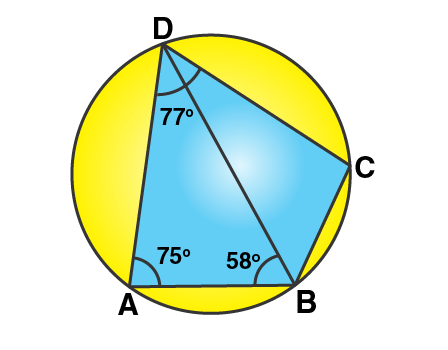Find:

(i) ∠BDC,

(ii) ∠BCD,

(iii) ∠BCA.

Solution: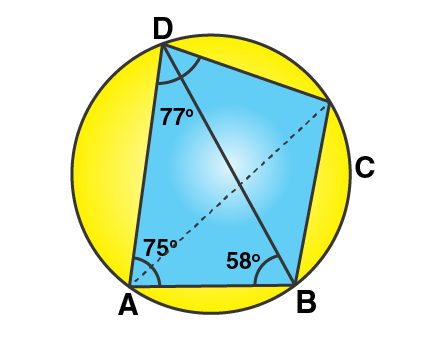(i) By angle sum property of triangle ABD,

∠ADB = 180o – 75o – 58o = 47o

Thus, ∠BDC = ∠ADC – ∠ADB = 77o – 47o = 30o

(ii) ∠BAD + ∠BCD = 180o

[Sum of opposite angles of a cyclic quadrilateral is 180o]

Thus, ∠BCD = 180o – 75o = 105o

(iii) ∠BCA = ∠ADB = 47o

[Angles subtended by the same chord on the circle are equal]

11. In the figure given below, O is the centre of the circle and triangle ABC is equilateral.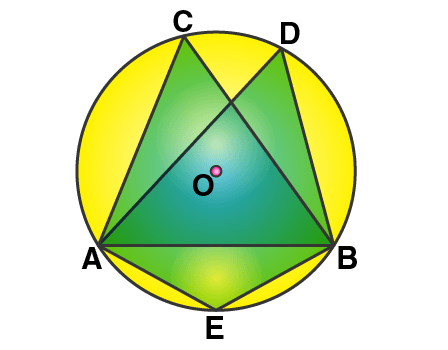Find: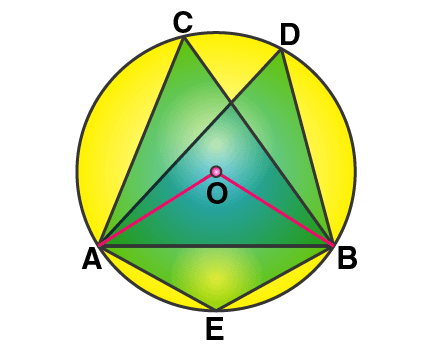Solution:

(i) As, it’s seen that ∠ACB and ∠ADB are in the same segment,

So,

(ii) Now, join OA and OB.

And, we have

∠AEB = ½ Reflex (∠AOB) = ½ (360o – 120o) = 120o

[Angle at the center is double the angle at the circumference subtend by the same chord]

12. Given: ∠CAB = 75o and ∠CBA = 50o. Find the value of ∠DAB + ∠ABD.

Solution: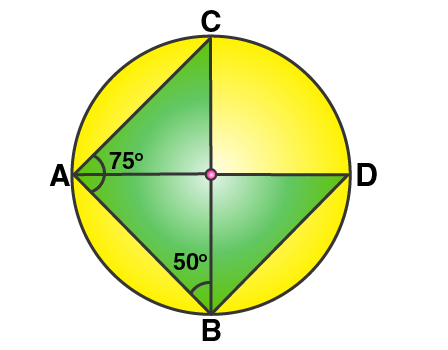Given, ∠CAB = 75o and ∠CBA = 50o

In ∆ABC, by angle sum property we have

∠ACB = 180o – (∠CBA + ∠CAB)

= 180o – (50o + 75o) = 180o – 125o

= 55o

And,

[Angles subtended by the same chord on the circle are equal]

Now, taking ∆ABD

∠DAB + ∠ABD + ∠ADB = 180o

∠DAB + ∠ABD + 55o = 180o

∠DAB + ∠ABD = 180o – 55o

∠DAB + ∠ABD = 125o

13. ABCD is a cyclic quadrilateral in a circle with centre O. If ∠ADC = 130o, find ∠BAC.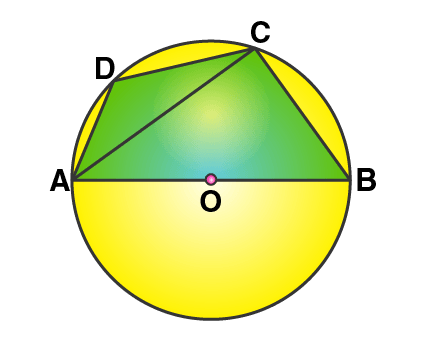Solution:

From the fig. its seem that,

∠ACB = 90o [Angle in a semi-circle is 90o]

Also,

∠ABC = 180o – ∠ADC = 180o – 130o = 50o

[Pair of opposite angles in a cyclic quadrilateral are supplementary]

By angle sum property of the right triangle ACB, we have

∠BAC = 90o – ∠ABC

= 90o – 50o

Thus, ∠BAC = 40o

14. In the figure given alongside, AOB is a diameter of the circle and ∠AOC = 110o, find ∠BDC.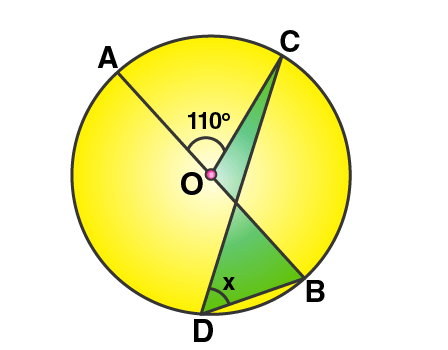Solution: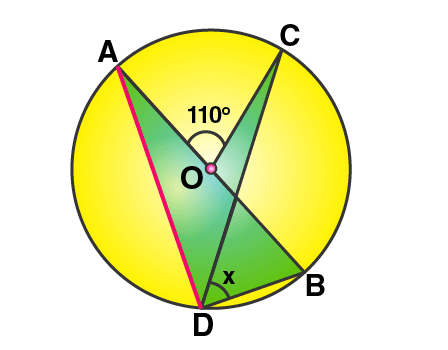So, we have

∠ADC = ½ ∠AOC = ½ x 110o = 55o

[Angle at the centre is double the angle at the circumference subtended by the same chord]

Also, we know that

[Angle in the semi-circle is a right angle]

Therefore,

∠BDC = 90o – ∠ADC = 90o – 55o

∠BDC = 35o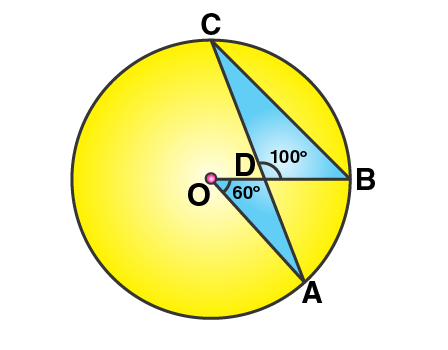15. In the following figure, O is the centre of the circle;AOB = 60o and BDC = 100o, find ∠OBC.

Solution:

Form the figure, we have

∠ACB = ½ ∠AOB = ½ x 60o = 30o

[Angle at the centre is double the angle at the circumference subtended by the same chord]

Now, by applying angle sum property in ∆BDC,

∠DBC = 180o – 100o – 30o = 50o

Therefore,

∠OBC = 50o

16. In ABCD is a cyclic quadrilateral in which ∠DAC = 27o, ∠DBA = 50o and ∠ADB = 33o. Calculate (i) ∠DBC, (ii) ∠DCB, (iii) ∠CAB.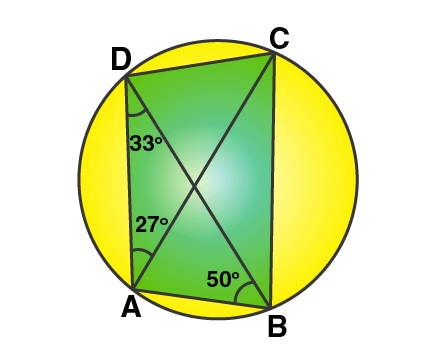Solution:

(i) It’s seen that,

∠DBC = ∠DAC = 27o

[Angles subtended by the same chord on the circle are equal]

(ii) It’s seen that,

And,

∠ACD = ∠ABD = 50o

[Angles subtended by the same chord on the circle are equal]

Thus,

∠DCB = ∠ACD + ∠ACB = 50o + 33o = 83o

∠DAB + ∠DCB = 180o

27o + ∠CAB + 83o = 180o

Thus,

∠CAB = 180o – 110o = 70o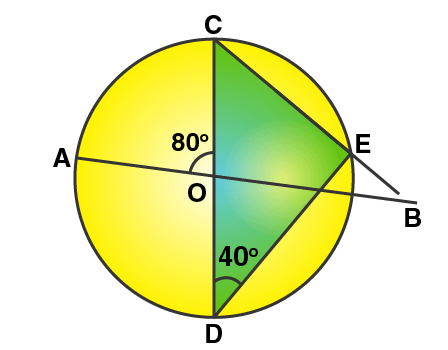17. In the figure given alongside, AB and CD are straight lines through the centre O of a circle. If ∠AOC = 80o and ∠CDE = 40o. Find the number of degrees in: (i) ∠DCE; (ii) ∠ABC.

Solution:

(i) Form the fig. its seen that,

∠DCE = 90o – ∠CDE = 90o – 40o = 50o

Therefore,

∠DEC = ∠OCB = 50o

(ii) In ∆BOC, we have

∠AOC = ∠OCB + ∠OBC [Exterior angle property of a triangle]

∠OBC = 80o – 50o = 30o  [Given ∠AOC = 80o]

Therefore, ∠ABC = 30o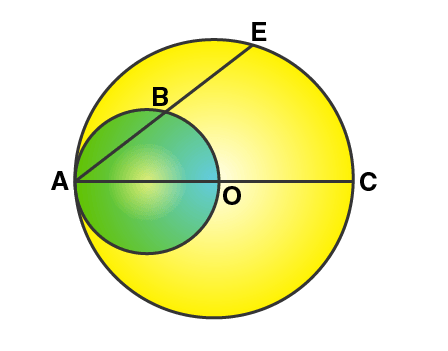18. In the figure given below, AC is a diameter of a circle, whose centre is O. A circle is described on AO as diameter. AE, a chord of the larger circle, intersects the smaller circle at B. Prove that AB = BE.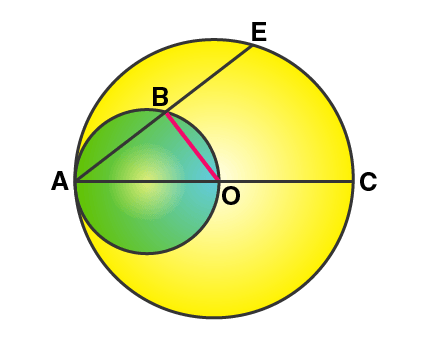Solution:

Firstly, join OB.

Then, ∠OBA = 90o [Angle in a semi-circle is a right angle]

That is, OB is perpendicular to AE.

Now, we know that the perpendicular draw from the centre to a chord bisects the chord.

Therefore,

AB = BE

19. (a) In the following figure,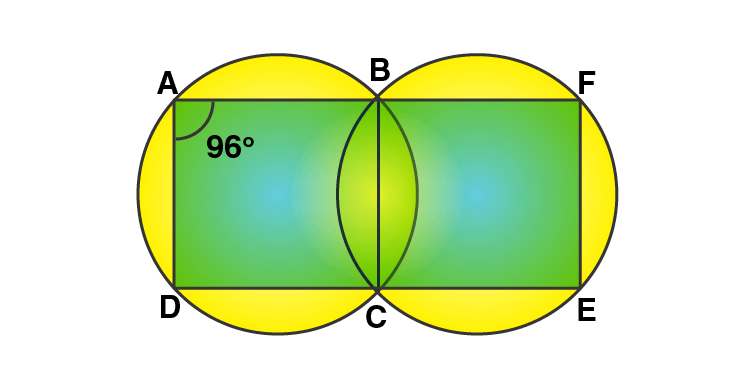(i) if ∠BAD = 96o, find ∠BCD and ∠BFE.

(ii) Prove that AD is parallel to FE.

(b) ABCD is a parallelogram. A circle

Solution:

(i) ABCD is a cyclic quadrilateral

So, ∠BAD + ∠BCD = 180o

[Pair of opposite angles in a cyclic quadrilateral are supplementary]

∠BCD = 180o – 96o = 84o

And, ∠BCE = 180o – 84o = 96o [Linear pair of angles]

Similarly, BCEF is a cyclic quadrilateral

So, ∠BCE + ∠BFE = 180o

[Pair of opposite angles in a cyclic quadrilateral are supplementary]

∠BFE = 180o – 96o = 84o

(ii) Now, ∠BAD + ∠BFE = 96o + 84o = 180o

But these two are interior angles on the same side of a pair of lines AD and FE.

20. Prove that:

(i) the parallelogram, inscribed in a circle, is a rectangle.

(ii) the rhombus, inscribed in a circle, is a square.

Solution: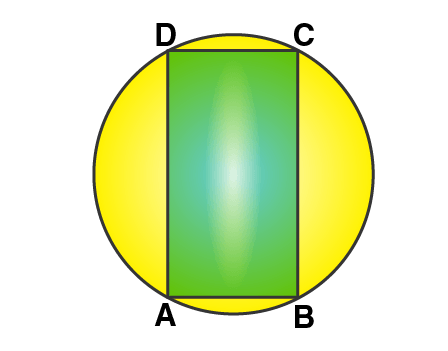(i) Let’s assume that ABCD is a parallelogram which is inscribed in a circle.

So, we have

∠BAD = ∠BCD [Opposite angles of a parallelogram are equal]

And ∠BAD + ∠BCD = 180o

[Pair of opposite angles in a cyclic quadrilateral are supplementary]

Thus, ∠BAD = ∠BCD = 90o

Similarly, the remaining two angles are 90o each and pair of opposite sides are equal.

Therefore,

ABCD is a rectangle.

– Hence Proved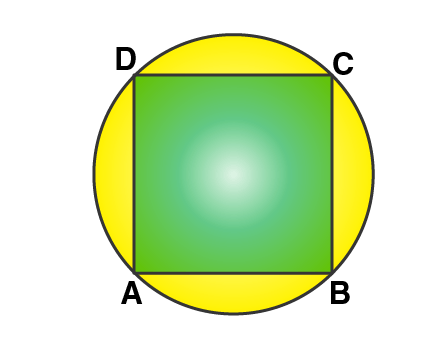(ii) Let’s assume that ABCD is a rhombus which is inscribed in a circle.

So, we have

∠BAD = ∠BCD [Opposite angles of a rhombus are equal]

And ∠BAD + ∠BCD = 180o

[Pair of opposite angles in a cyclic quadrilateral are supplementary]

Thus, ∠BAD = ∠BCD = 90o

Similarly, the remaining two angles are 90o each and all the sides are equal.

Therefore,

ABCD is a square.

– Hence Proved

21. In the following figure, AB = AC. Prove that DECB is an isosceles trapezium.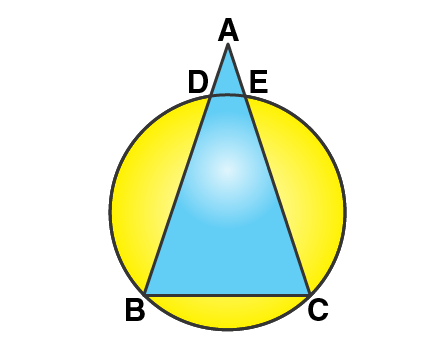Solution:

Give, AB = AC

So, ∠B = ∠C … (1)

[Angles opposite to equal sides are equal]

And, DECB is a cyclic quadrilateral.

So, ∠B + ∠DEC = 180o

[Pair of opposite angles in a cyclic quadrilateral are supplementary]

∠C + ∠DEC = 180o …. (Using 1)

But this is the sum of interior angles on one side of a transversal.

DE || BC.

But, ∠ADE = ∠B and ∠AED = ∠C [Corresponding angles]

AB – AD = AC = AE [As AB = AC]

BD = CE

Hence, we have DE || BC and BD = CE

Therefore,

DECB is an isosceles trapezium.

22. Two circles intersect at P and Q. Through P diameters PA and PB of the two circles are drawn. Show that the points A, Q and B are collinear.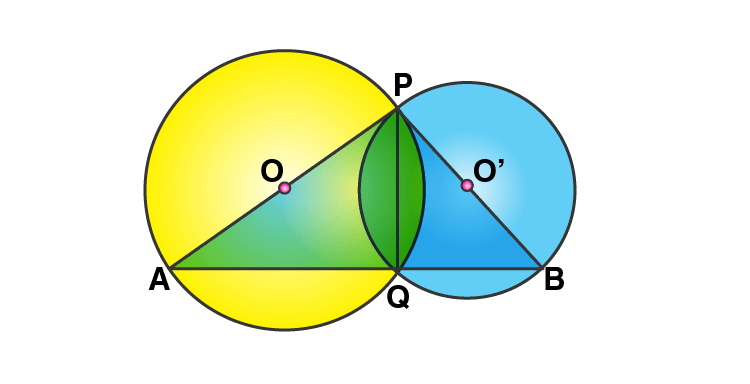Solution:

Let O and O’ be the centres of two intersecting circles, where points of the intersection are P and Q and PA and PB are their diameters respectively.

Join PQ, AQ and QB.

Thus, ∠AQP = 90o and ∠BQP = 90o

[Angle in a semicircle is a right angle]

Now, adding both these angles we get

∠AQP + ∠BQP = 180o

∠AQB = 180o

Therefore, the points A, Q and B are collinear.

23. The figure given below, shows a circle with centre O. Given: AOC = a and ABC = b.

(i) Find the relationship between a and b

(ii) Find the measure of angle OAB, if OABC is a parallelogram.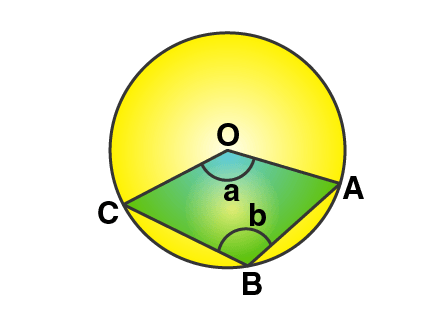Solution:

(i) It’s seen that,

∠ABC = ½ Reflex (∠COA)

[Angle at the centre is double the angle at the circumference subtended by the same chord]

So, b = ½ (360o – a)

a + 2b = 180o ….. (1)

(ii) As OABC is a parallelogram, the opposite angles are equal.

So, a = b

Now, using the above relationship in (1)

3a = 180o

a = 60o

Also, OC || BA

∠COA + ∠OAB = 180o

60o + ∠OAB = 180o

Therefore,

∠OAB = 120o

24. Two chords AB and CD intersect at P inside the circle. Prove that the sum of the angles subtended by the arcs AC and BD as the center O is equal to twice the angle APC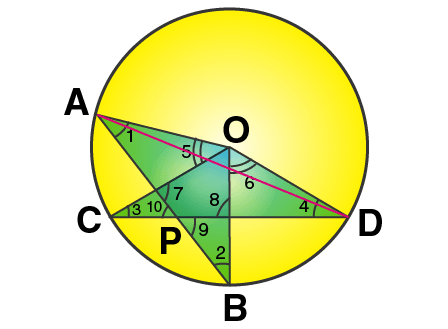Solution:

Required to prove: ∠AOC + ∠BOD = 2∠APC

OA, OB, OC and OD are joined.

Now, it’s seen that

[Angle at the centre is double the angle at the circumference subtended by the same chord]

Similarly,

Adding (1) and (2), we have

So, from (3) and (4) we have

∠AOC + ∠BOD = 2∠APC

25. In the figure given RS is a diameter of the circle. NM is parallel to RS and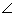MRS = 29o

Calculate: (i) ∠RNM; (ii) ∠NRM.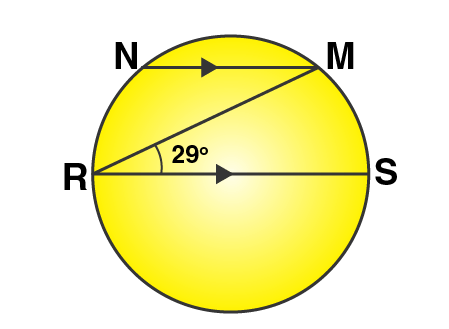Solution: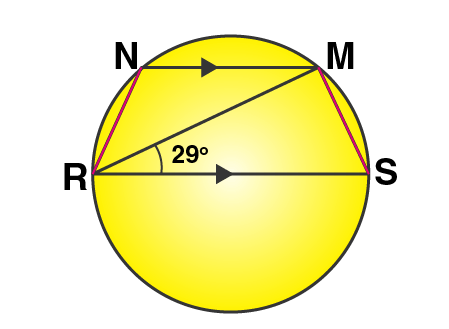(i) Join RN and MS

∠RMS = 90o [Angle in a semi-circle is a right angle]

So, by angle sum property of ∆RMS

∠RMS = 90o – 29o = 61o

And,

∠RNM = 180o – ∠RSM = 180o – 61o = 119o

[Pair of opposite angles in a cyclic quadrilateral are supplementary]

(ii) Now as RS || NM,

∠NMR = ∠MRS = 29o [Alternate angles]

∠NMS = 90o + 29o = 119o

Also, we know that

∠NRS + ∠NMS = 180o

[Pair of opposite angles in a cyclic quadrilateral are supplementary]

∠NRM + 29o + 119o = 180o

∠NRM = 180o – 148o

Therefore,

∠NRM = 32o

26. In the figure given alongside, AB || CD and O is the center of the circle. If ADC = 25o; find the angle AEB. Give reasons in support of your answer.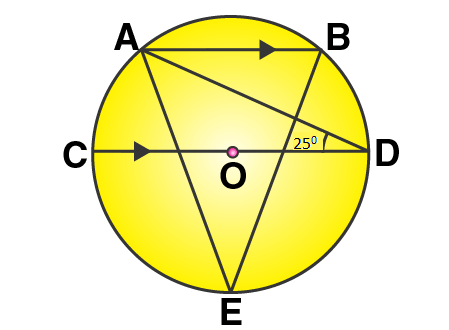Solution: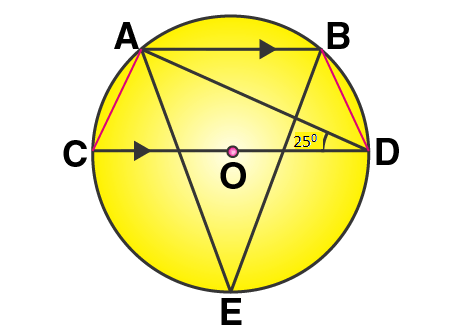Join AC and BD.

So, we have

∠CAD = 90o and ∠CBD = 90o

[Angle is a semicircle is a right angle]

And, AB || CD

Thus,

∠ADB = 180o – 25o – ∠BAC = 180o – 25o – 115o = 40o

[Pair of opposite angles in a cyclic quadrilateral are supplementary]

Finally,

[Angles subtended by the same chord on the circle are equal]

27. Two circles intersect at P and Q. Through P, a straight line APB is drawn to meet the circles in A and B. Through Q, a straight line is drawn to meet the circles at C and D. Prove that AC is parallel to BD.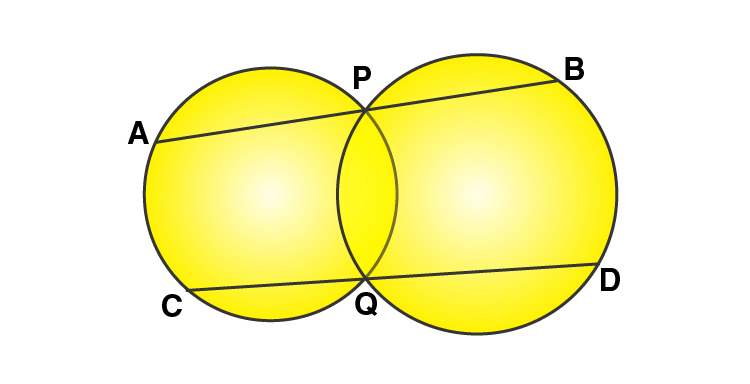Solution: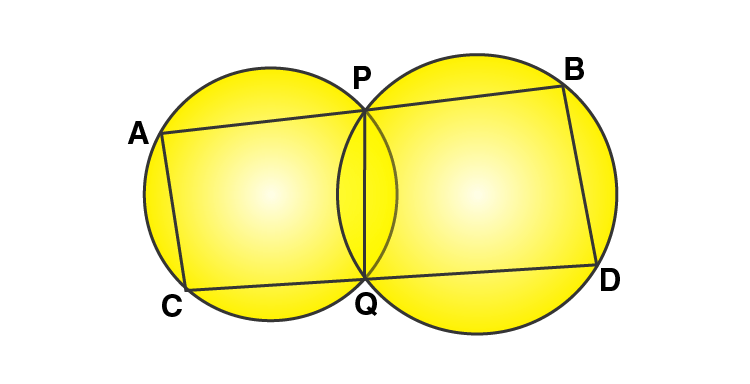Let’s join AC, PQ and BD.

As ACQP is a cyclic quadrilateral

∠CAP + ∠PQC = 180o ……. (i)

[Pair of opposite angles in a cyclic quadrilateral are supplementary]

Similarly, as PQDB is a cyclic quadrilateral

∠PQD + ∠DBP = 180o ……. (ii)

Again, ∠PQC + ∠PQD = 180o …… (iii) [Linear pair of angles]

Using (i), (ii) and (iii) we have

∠CAP + ∠DBP = 180o

Or ∠CAB + ∠DBA = 180o

We know that, if the sum of interior angles between two lines when intersected by a transversal are supplementary.

Then, AC || BD.

28. ABCD is a cyclic quadrilateral in which AB and DC on being produced, meet at P such that PA = PD. Prove that AD is parallel to BC.

Solution: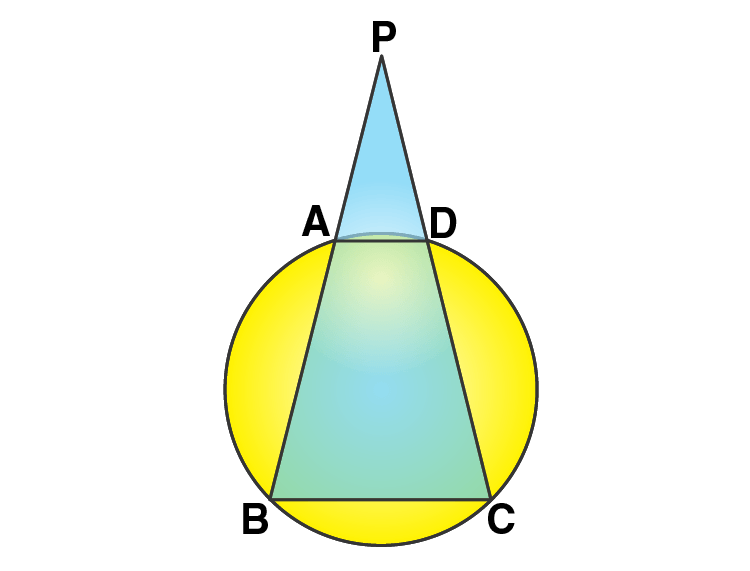Let’s assume that ABCD be the given cyclic quadrilateral.

Also, PA = PD [Given]

So, ∠PAD = ∠PDA …… (1)

[Angles opposite to equal sides are equal]

And,

Similarly,

∠CDA = 180o – ∠PDA = 180o – ∠PAD [From (1)]

As the opposite angles of a cyclic quadrilateral are supplementary,

∠ABC = 180o – ∠CDA = 180o – (180o – ∠PAD) = ∠PAD

Thus,

∠ABC = ∠DCB = ∠PAD = ∠PDA

Which is only possible when AD || BC.

Exercise 17(B) Page No: 265

1. In a cyclic-trapezium, the non-parallel sides are equal and the diagonals are also equal.

Prove it.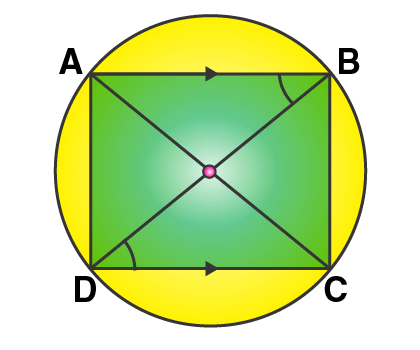Solution:

Let ABCD be the cyclic trapezium in which AB || DC, AC and BD are the diagonals.

Required to prove:

(ii) AC = BD

Proof:

It’s seen that chord AD subtends ∠ABD and chord BC subtends ∠BDC at the circumference of the circle.

But, ∠ABD = ∠BDC [Alternate angles, as AB || DC with BD as the transversal]

So, Chord AD must be equal to chord BC

DC = DC [Common]

∠CAD = ∠CBD [Angles in the same segment are equal]

Hence, by SAS criterion of congruence

Therefore, by CPCT

AC = BD

2. In the following figure, AD is the diameter of the circle with centre O. Chords AB, BC and CD are equal. If ∠DEF = 110o, calculate:

(i) ∠AFE, (ii) ∠FAB.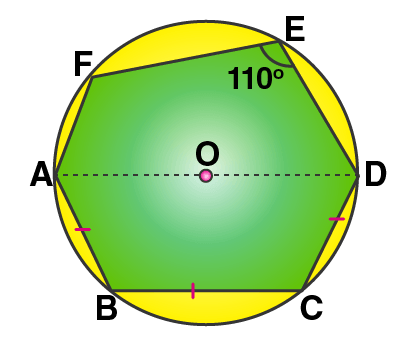Solution:

Join AE, OB and OC.

(i) As AOD is the diameter

∠AED = 90o [Angle in a semi-circle is a right angle]

But, given ∠DEF = 110o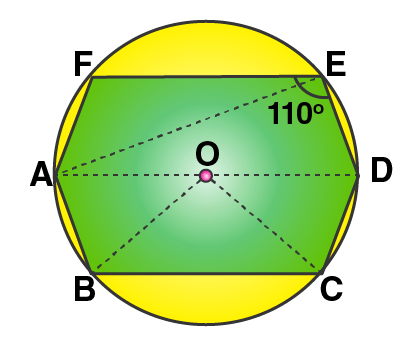So,

∠AEF = ∠DEF – ∠AED = 110o – 90o = 20o

(ii) Also given, Chord AB = Chord BC = Chord CD

So,

∠AOB = ∠BOC = ∠COD [Equal chords subtends equal angles at the centre]

But,

∠AOB + ∠BOC + ∠COD = 180o [Since, AOD is a straight line]

Thus,

∠AOB = ∠BOC = ∠COD = 60o

Now, in ∆OAB we have

OA = OB [Radii of same circle]

So, ∠OAB = ∠OBA [Angles opposite to equal sides]

But, by angle sum property of ∆OAB

∠OAB + ∠OBA = 180o – ∠AOB

= 180o – 60o

= 120o

Therefore, ∠OAB = ∠OBA = 60o

∠DEF + ∠DAF = 180o

∠DAF = 180o – ∠DEF

= 180o – 110o

= 70o

Thus,

∠FAB = ∠DAF + ∠OAB

= 70o + 60o = 130o

3. If two sides of a cycli-quadrilateral are parallel; prove that:

(i) its other two sides are equal.

(ii) its diagonals are equal.

Solution:

Let ABCD is a cyclic quadrilateral in which AB || DC. AC and BD are its diagonals.

Required to prove: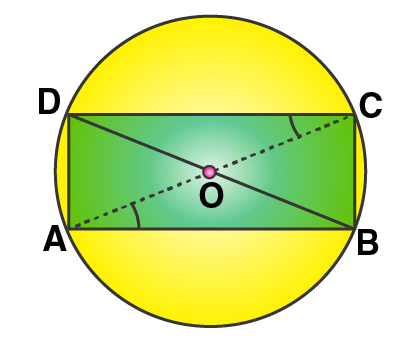(i) AD = BC

(ii) AC = BD

Proof:

(i) As AB || DC (given)

∠DCA = ∠CAB [Alternate angles]

Now, chord AD subtends ∠DCA and chord BC subtends ∠CAB at the circumference of the circle.

So,

∠DCA = ∠CAB

(ii) Now, in ∆ABC and ∆ADB

AB = AB [Common]

∠ACB = ∠ADB [Angles in the same segment are equal]

Hence, by SAS criterion of congruence

Therefore, by CPCT

AC = BD

4. The given figure show a circle with centre O. Also, PQ = QR = RS and ∠PTS = 75°.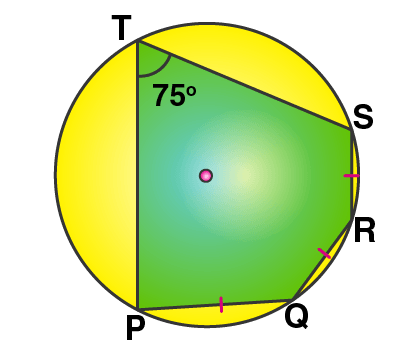Calculate:

(i) ∠POS,

(ii) ∠QOR,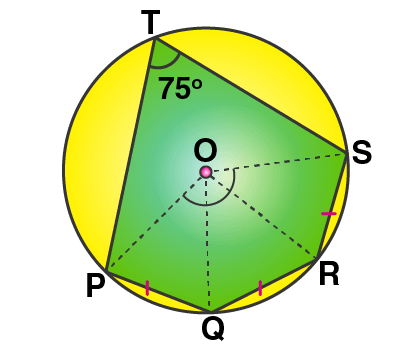(iii) ∠PQR.

Solution:

Join OP, OQ, OR and OS.

Given, PQ = QR = RS

So, ∠POQ = ∠QOR = ∠ROS [Equal chords subtends equal angles at the centre]

Arc PQRS subtends ∠POS at the centre and ∠PTS at the remaining part of the circle.

Thus,

∠POS = 2 x ∠PTS = 2 x 75o = 150o

∠POQ + ∠QOR + ∠ROS = 150o

∠POQ = ∠QOR = ∠ROS = 150o/ 3 = 50o

In ∆OPQ we have,

OP = OQ [Radii of the same circle]

So, ∠OPQ = ∠OQP [Angles opposite to equal sides are equal]

But, by angle sum property of ∆OPQ

∠OPQ + ∠OQP + ∠POQ = 180o

∠OPQ + ∠OQP + 50o = 180o

∠OPQ + ∠OQP = 130o

2 ∠OPQ = 130o

∠OPQ = ∠OPQ = 130o/ 2 = 65o

Similarly, we can prove that

In ∆OQR,

∠OQR = ∠ORQ = 65o

And in ∆ORS,

∠ORS = ∠OSR = 65o

Hence,

(i) ∠POS = 150o

(ii) ∠QOR = 50o and

(iii) ∠PQR = ∠PQO + ∠OQR = 65o + 65o = 130o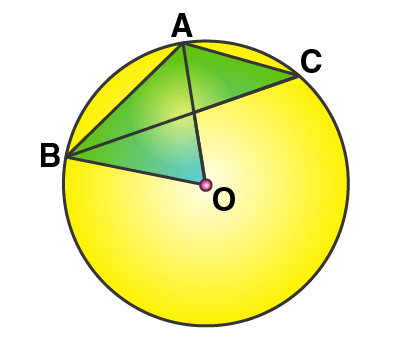5. In the given figure, AB is a side of a regular six-sided polygon and AC is a side of a regular eight-sided polygon inscribed in the circle with centre O. calculate the sizes of:

(i) AOB,

(ii) ACB,

(iii) ABC.

Solution:

(i) Arc AB subtends ∠AOB at the centre and ∠ACB at the remaining part of the circle.

∠ACB = ½ ∠AOB

And as AB is the side of a regular hexagon, we have

∠AOB = 60o

(ii) Now,

∠ACB = ½ (60o) = 30o

(iii) Since AC is the side of a regular octagon,

∠AOC = 360o/ 8 = 45o

Again, arc AC subtends ∠AOC at the centre and ∠ABC at the remaining part of the circle.

∠ABC = ½ ∠AOC

∠ABC = 45o/ 2 = 22.5o

Exercise 17(C) Page No: 265

1. In the given circle with diameter AB, find the value of x.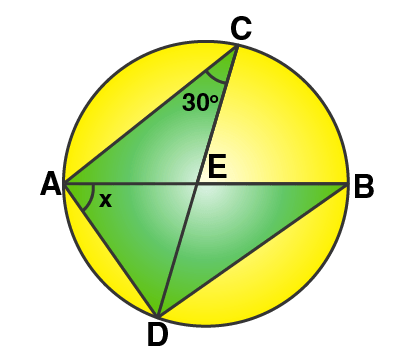Solution:

Now,

∠ABD = ∠ACD = 30o [Angles in the same segment]

In ∆ADB, by angle sum property we have

But, we know that angle in a semi-circle is 90o

So,

x + 90o + 30o = 180o

x = 180o – 120o

Hence, x = 60o

2. In the given figure, ABC is a triangle in which ∠BAC = 30o. Show that BC is equal to the radius of the circum-circle of the triangle ABC, whose center is O.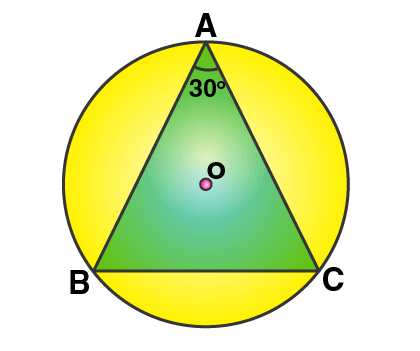Solution:

Firstly, join OB and OC.

Proof:

∠BOC = 2∠BAC = 2 x 30o = 60o

Now, in ∆OBC

OB = OC [Radii of same circle]

So, ∠OBC = ∠OCB [Angles opposite to equal sides]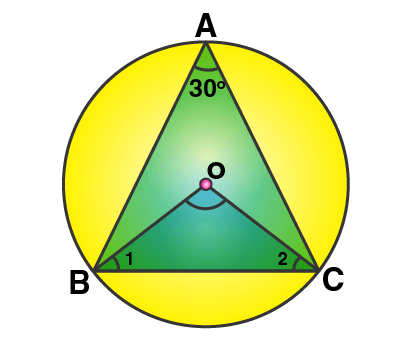And in ∆OBC, by angle sum property we have

∠OBC + ∠OCB + ∠BOC = 180o

∠OBC + ∠OBC + 60o = 180o

2 ∠OBC = 180o – 60o = 120o

∠OBC = 120o/ 2 = 60o

So, ∠OBC = ∠OCB = ∠BOC = 60o

Thus, ∆OBC is an equilateral triangle.

So,

BC = OB = OC

But, OB and OC are the radii of the circum-circle.

Therefore, BC is also the radius of the circum-circle.

3. Prove that the circle drawn on any one of the equal sides of an isosceles triangle as diameter bisects the base.

Solution: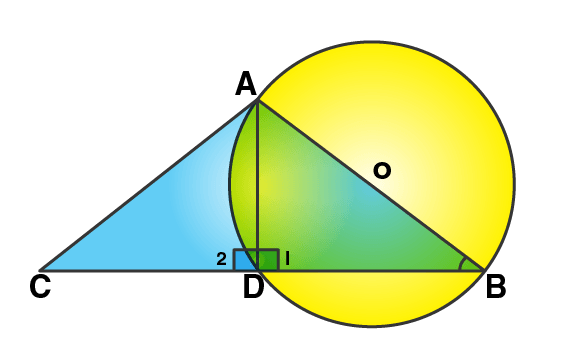Let’s consider ∆ABC, AB = AC and circle with AB as diameter is drawn which intersects the side BC and D.

Proof:

It’s seen that,

∠ADB = 90o [Angle in a semi-circle]

And,

Now, in right ∆ABD and ∆ACD

AB = AC [Given]

Hence, by R.H.S criterion of congruence.

∆ABD ≅ ∆ACD

Now, by CPCT

BD = DC

Therefore, D is the mid-point of BC.

4. In the given figure, chord ED is parallel to diameter AC of the circle. Given ∠CBE = 65o, calculate ∠DEC.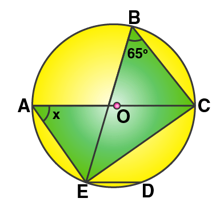Solution: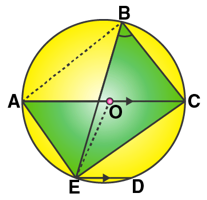Join OE.

Arc EC subtends ∠EOC at the centre and ∠EBC at the remaining part of the circle.

∠EOC = 2∠EBC = 2 x 65o = 130o

Now, in ∆OEC

OE = OC [Radii of the same circle]

So, ∠OEC = ∠OCE

But, in ∆EOC by angle sum property

∠OEC + ∠OCE + ∠EOC = 180o [Angles of a triangle]

∠OCE + ∠OCE + ∠EOC = 180o

2 ∠OCE + 130o = 180o

2 ∠OCE = 180o – 130o

∠OCE = 50o/ 2 = 25o

And, AC || ED [Given]

∠DEC = ∠OCE [Alternate angles]

Thus,

∠DEC = 25o

5. The quadrilateral formed by angle bisectors of a cyclic quadrilateral is also cyclic. Prove it.

Solution: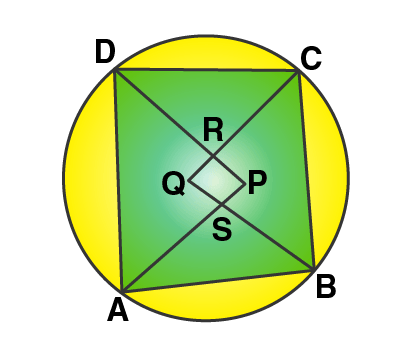Let ABCD be a cyclic quadrilateral and PQRS be the quadrilateral formed by the angle bisectors of angle ∠A, ∠B, ∠C and ∠D.

Required to prove: PQRS is a cyclic quadrilateral.

Proof:

By angle sum property of a triangle

In ∆APD,

And, in ∆BQC

∠QBC + ∠BCQ + ∠BQC = 180o …. (ii)

Adding (i) and (ii), we get

∠PAD + ∠ADP + ∠APD + ∠QBC + ∠BCQ + ∠BQC = 180o + 180o = 360o …… (iii)

But,

∠PAD + ∠ADP + ∠QBC + ∠BCQ = ½ [∠A + ∠B + ∠C + ∠D]

= ½ x 360o = 180o

Therefore,

∠APD + ∠BQC = 360o – 180o = 180o [From (iii)]

But, these are the sum of opposite angles of quadrilateral PRQS.

Therefore,

6. In the figure, ∠DBC = 58°. BD is a diameter of the circle. Calculate:

(i) ∠BDC

(ii) ∠BEC

(iii) ∠BAC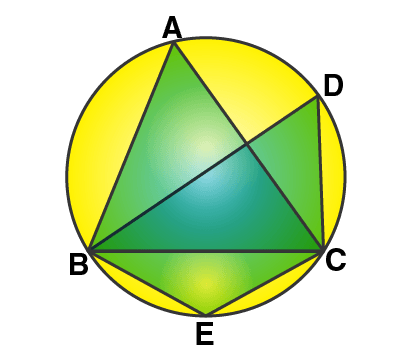Solution:

(i) Given that BD is a diameter of the circle.

And, the angle in a semicircle is a right angle.

So, ∠BCD = 90°

Also given that,

∠DBC = 58°

In ∆BDC,

∠DBC + ∠BCD + ∠BDC = 180o

58° + 90° + ∠BDC = 180o

148o + ∠BDC = 180o

∠BDC = 180o – 148o

Thus, ∠BDC = 32o

(ii) We know that, the opposite angles of a cyclic quadrilateral are supplementary.

∠BEC + ∠BDC = 180o

∠BEC + 32o = 180o

∠BEC = 148o

∠BAC + ∠BEC = 180o [Opposite angles of a cyclic quadrilateral are supplementary]

∠BAC + 148o = 180o

∠BAC = 180o – 148o

Thus, ∠BAC = 32o

7. D and E are points on equal sides AB and AC of an isosceles triangle ABC such that AD = AE. Prove that the points B, C, E and D are concyclic.

Solution:

Given,

∆ABC, AB = AC and D and E are points on AB and AC such that AD = AE.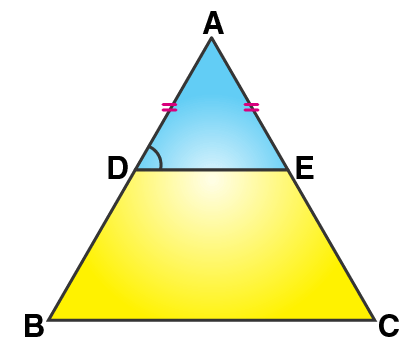And, DE is joined.

Required to prove: Points B, C, E and D are concyclic

Proof:

In ∆ABC,

AB = AC [Given]

So, ∠B = ∠C [Angles opposite to equal sides]

Similarly,

So, ∠ADE = ∠AED [Angles opposite to equal sides]

Now, in ∆ABC we have

Hence, DE || BC [Converse of BPT]

So,

(180o – ∠EDB) = ∠B

∠B + ∠EDB = 180o

But, it’s proved above that

∠B = ∠C

So,

∠C + ∠EDB = 180o

Thus, opposite angles are supplementary.

Similarly,

∠B + ∠CED = 180o

Hence, B, C, E and D are concyclic.

8. In the given figure, ABCD is a cyclic quadrilateral. AF is drawn parallel to CB and DA is produced to point E. If ADC = 92o, FAE = 20o; determine BCD. Given reason in support of your answer.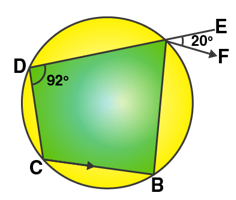Solution:

Given,

AF || CB and DA is produced to E such that ∠ADC = 92o and ∠FAE = 20o

So,

∠B + ∠D = 180o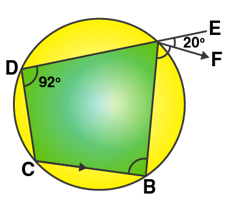∠B + 92o = 180o

∠B = 88o

As AF || CB, ∠FAB = ∠B = 88o

Ext. ∠BAE = ∠BAF + ∠FAE

= 88o + 22o = 108o

But, Ext. ∠BAE = ∠BCD

Therefore,

∠BCD = 108o

9. If I is the incentre of triangle ABC and AI when produced meets the circumcircle of triangle ABC in point D. If ∠BAC = 66o and ∠ABC = 80o. Calculate:

(i) ∠DBC,

(ii) ∠IBC,

(iii) ∠BIC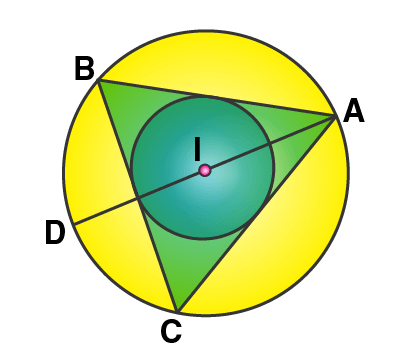Solution: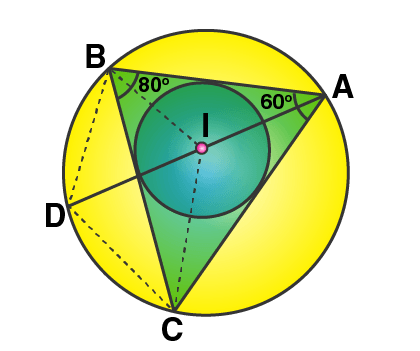Join DB and DC, IB and IC.

Given, if ∠BAC = 66o and ∠ABC = 80o, I is the incentre of the ∆ABC.

(i) As it’s seen that ∠DBC and ∠DAC are in the same segment,

So, ∠DBC = ∠DAC

But, ∠DAC = ½ ∠BAC = ½ x 66o = 33o

Thus, ∠DBC = 33o

(ii) And, as I is the incentre of ∆ABC, IB bisects ∠ABC.

Therefore,

∠IBC = ½ ∠ABC = ½ x 80o = 40o

(iii) In ∆ABC, by angle sum property

∠ACB = 180o – (∠ABC + ∠BAC)

∠ACB = 180o – (80o + 66o)

∠ACB = 180o – 156o

∠ACB = 34o

And since, IC bisects ∠C

Thus, ∠ICB = ½ ∠C = ½ x 34o = 17o

Now, in ∆IBC

∠IBC + ∠ICB + ∠BIC = 180o

40o + 17o + ∠BIC = 180o

57o + ∠BIC = 180o

∠BIC = 180o – 57o

Therefore, ∠BIC = 123o

10. In the given figure, AB = AD = DC = PB and ∠DBC = xo. Determine, in terms of x:

(i) ∠ABD, (ii) ∠APB.

Hence or otherwise, prove that AP is parallel to DB.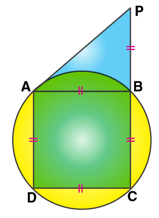Solution: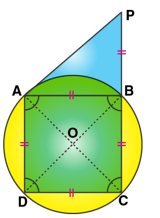Given, AB = AD = DC = PB and ∠DBC = xo

Join AC and BD.

Proof:

∠DAC = ∠DBC = xo [Angles in the same segment]

And, ∠DCA = ∠DAC = xo [As AD = DC]

Also, we have

∠ABD = ∠DAC [Angles in the same segment]

And, in ∆ABP

Ext. ∠ABD = ∠BAP + ∠APB

But, ∠BAP = ∠APB [Since, AB = BP]

2 xo = ∠APB + ∠APB = 2∠APB

2∠APB = 2xo

So, ∠APB = xo

Thus, ∠APB = ∠DBC = xo

But these are corresponding angles,

Therefore, AP || DB.

11. In the given figure; ABC, AEQ and CEP are straight lines. Show that ∠APE and ∠CQE are supplementary.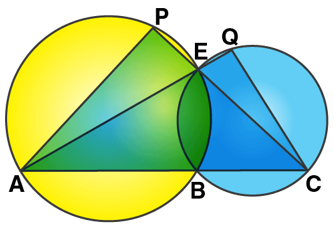Solution: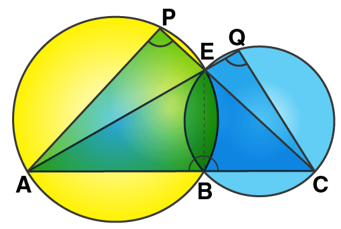Join EB.

∠APE + ∠ABE = 180o ….. (i) [Opposite angles of a cyclic quad. are supplementary]

∠CQE + ∠CBE = 180o ….. (ii) [Opposite angles of a cyclic quad. are supplementary]

Adding (i) and (ii), we have

∠APE + ∠ABE + ∠CQE + ∠CBE = 180o + 180o = 360o

∠APE + ∠ABE + ∠CQE + ∠CBE = 360o

But, ∠ABE + ∠CBE = 180o [Linear pair]

∠APE + ∠CQE + 180o = 360o

∠APE + ∠CQE = 180o

Therefore, ∠APE and ∠CQE are supplementary.

12. In the given, AB is the diameter of the circle with centre O.

If ∠ADC = 32o, find angle BOC.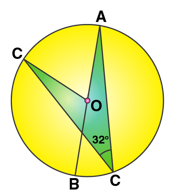Solution:

Arc AC subtends ∠AOC at the centre and ∠ADC at the remaining part of the circle.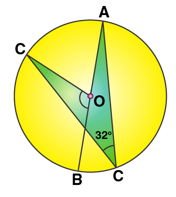Thus, ∠AOC = 2∠ADC

∠AOC = 2 x 32o = 64o

As ∠AOC and ∠BOC are linear pair, we have

∠AOC + ∠BOC = 180o

64o + ∠BOC = 180o

∠BOC = 180o – 64o

Therefore, ∠BOC = 116o

The given solutions are as per the 2019-20 Concise Selina textbook. The Selina Solutions for the academic year 2020-21 will be updated soon.